# 12v Solar battery monitor

• Hi
I have installed a node to monitor our 12v auxiliary supply for our home. The power system consists of a 200w panel on the roof and a 105AH battery located in our server cabinet. When we built our home I ran a 12v Backbone as well so we can access the low voltage power all over . To date it has been used to run a system of night lights and reading lamps but now that I have found Mysensors I hope it will soon be powering a lot more.

Ok this node has been running for a few weeks now so thought i would share it with others. it monitors battery voltage, Charge current, Dis-charge current, Ambient temp and battery Temp. I have included the temperature monitors after a friend had his battery develop an internal short and melt in his caravan, not a pretty sight.

My notes are based on my breadboard version which was built around an Arduino nano, In the final I used a Pro Mini.

Parts List:

1 x Arduino I have used a Nano but other types or OK too
1 x ACS712 Current sensing Module. These are available in 5A, 20A and 30A. Choose whichever current fills your needs. Only one line of code needs to be modified to suit your selected device.
2 x DS18b20 Temperature sensors
1 x Voltage sensing module or 2 x resistors to be used as a voltage divider. I have used a module that has a maximum reading of 25v. That means when the module sees 25v on its input the output of the module will be 5v.
1 x nRF24L01+ 2.4ghz Transceiver
1 x LM2596 regulator module or some other regulator able to supply 5v
1x 330 ohm resistor
1x 4.7 k ohm resistor
1x LED
1x push button

When using the analog inputs of the Arduino it is very important that you have a very stable 5v supply voltage. The 20A ACS712 shows a change of only 100mv for every 1A so you can see even small fluctuations can cause sizable errors in your readings. During construction I found powering the circuit from the usb port to give unreliable results and opted for a dedicated 5v supply instead.

Construction:
Wire the devices as shown below.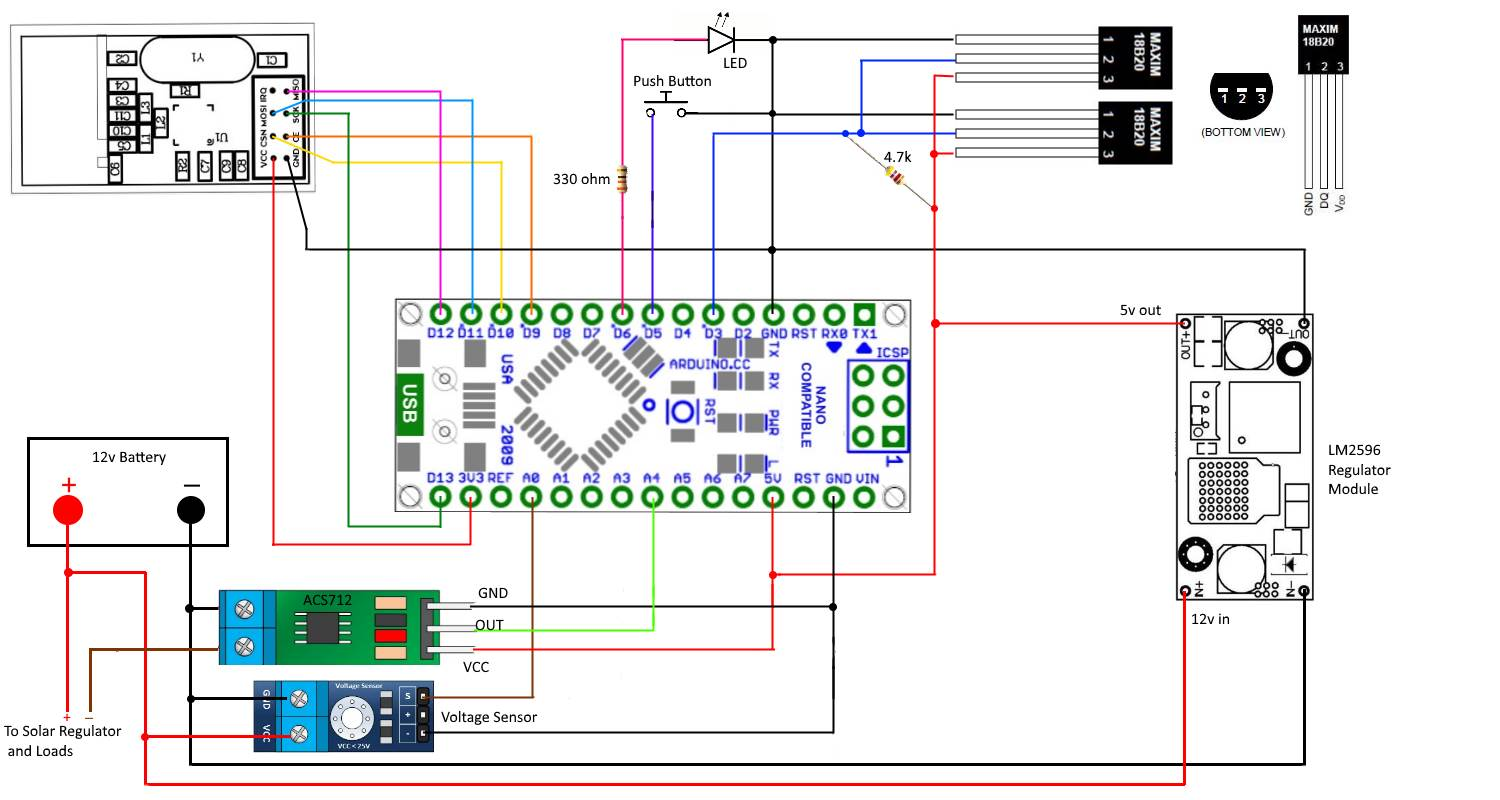The Sketch:

`````` /*Sketch for a MySensor node to monitor a 12v battery with a solar panel for charging
* The node monitors battery voltage,current into and out of the battery, ambient temperature and battery temperature.
* 2 x DS18b20 dallas temperature ic's their data pins connected to arduino digital pin 3
* 1 x ACS712 current sensor module connected to  arduino analog pin A4
* 1 x 25v voltage sensor module connected to arduino analog pin A0
* 1 x nRF24L01+  2.4ghz tranceiver connected as per the MySensors web site.
* 1 x LED connected via a 330 ohm resistor to pin 6
* 1 x push button connected to pin 5
*/

#include <MySensor.h>
#include <SPI.h>
#include <OneWire.h>
#include <DallasTemperature.h>

#define ONE_WIRE_BUS 3                       // Ds18b20 data wire is connected to digital pin 3 on the Arduino
#define ID_S_TEMPA 0                         // First temp device
#define ID_S_TEMPB 1                         // second temp device
#define ID_S_MULTIMETERV 3                   // Multimeter device for voltage measurement
#define ID_S_MULTIMETERC 4                   // Multimeter device for positive current measurement
#define ID_S_MULTIMETERC1 5                  // Multimeter device for negative current measurement
#define NUM_SAMPLES 10                       // number of analog voltage samples to take per reading

int ledPin = 6;                               // the pin for the LED
int buttonPin = 5;                            // the input pin for offset pushbutton
int buttonState = 0;                          // variable for reading the pin status
unsigned long SLEEP_TIME = 30000;            // Sleep time between reads (in milliseconds)
int lastmilli = 25000;                       // set to an arbitary number outside of expected current sensor range to ensure a change when first run
float sensitivity = 100 ;                    //change this to 185 for ACS712-5 or to 100 for ACS712-20A or to 66 for ACS712-30A
int VQ = 0;                                  //Placeholder for quiescent voltage calculations
int ACSPin = A4;                             // Analog pin number the ACS712 data pin connects to
float lastTemperature;                    //Array to hold the last temp readings sent to gateway, only send new data if different
int sum = 0;                                 // sum of voltage samples taken
unsigned char sample_count = 0;              // current sample number
int lastVoltage = 30000;                     // set to an arbitary number outside of expected voltage sensor range to ensure a change when first run
int voltagePin = A0;                         // analog pin voltage sensor or voltage divider is connected to
int voltSenseMax = 25000;                    // set to the maximum input voltage in millivolts of your voltage divider input
OneWire oneWire(ONE_WIRE_BUS);               // Setup a oneWire instance to communicate with any OneWire devices (not just Maxim/Dallas temperature ICs)
DallasTemperature sensors(&oneWire);         // Pass our oneWire reference to Dallas Temperature.

MySensor gw;

// ------ Initialize  messages -------
MyMessage msg(0,V_TEMP);
MyMessage msg_S_MULTIMETERv(ID_S_MULTIMETERV,V_VOLTAGE);
MyMessage msg_S_MULTIMETERc(ID_S_MULTIMETERC,V_CURRENT);
MyMessage msg_S_MULTIMETERc1(ID_S_MULTIMETERC1,V_CURRENT);

void setup()
{

sensors.begin();                                    // Start up the onewire library
gw.begin();                                         // Startup and initialize MySensors library. Set callback for incoming messages.
gw.sendSketchInfo("Battery Status Sensor", "1");    // Send the sketch version information to the gateway and Controller

// ------ Present all sensors to controller ------
gw.present(ID_S_TEMPA, S_TEMP);
gw.present(ID_S_TEMPB, S_TEMP);
gw.present(ID_S_MULTIMETERV,V_VOLTAGE);
gw.present(ID_S_MULTIMETERC,V_CURRENT);
gw.present(ID_S_MULTIMETERC1,V_CURRENT);

pinMode(buttonPin, INPUT_PULLUP);                     // Set buttonPin as input and turn on internal pull up resistor
pinMode(ledPin, OUTPUT);                              // Set ledPin as output
digitalWrite(ledPin, LOW);                            // Make sure ledPin is off

// ------ load offset for current sensor
if (validCheck == 120){                          // check to see if valid data exists
//  Serial.print(" positive VQ offset loaded..."); Serial.println(VQ);
}
else if (validCheck == 125) {
//  Serial.print(" negative VQ offset loaded..."); Serial.println(VQ);
}
else {
// Serial.println("VQ offset not set");
}

delay(500);
}

void loop()
{

//Serial.print("buttonstate..."); Serial.println(buttonState);
if (buttonState == LOW) {
VQ = determineVQ(ACSPin);                           //Returns the offset count needed to show zero with no load

if (VQ >= 0 && VQ < 255) {                              //check for valid data. VQ is positive number
gw.saveState(0, 120);                               // Store 120 value  in eeprom position 0. use this to check for valid data at boot
gw.saveState(1, VQ);                                // Store offset count in eeprom. in case of re-boot
}
else if (VQ < 0 && VQ > -255) {                              // VQ is a negative number. negatives cannot be stored in eeprom
gw.saveState(0, 125);                               // Store 125 value  in eeprom position 0. use this to check for valid data at boot
gw.saveState(1, abs(VQ));                                // convert VQ to positive and  Store offset count in eeprom. in case of re-boot
}

}

// ------------------ Start voltage readings --------------------

sample_count = 0;
sum = 0;
while (sample_count < NUM_SAMPLES) {                                   // take a number of voltage samples
sample_count++;
delay(10);
}
//Serial.print("sum count..."); Serial.println((sum / NUM_SAMPLES));      // print the count result. will be between 0 and 1023
int voltageI = map(sum/NUM_SAMPLES,0,1023,0,voltSenseMax);              // map the reading and get our result in millivolts
//Serial.print("mapped volts..."); Serial.println(voltageI / 1000.0, 1);  // convert millivolts back to volts and print. the 1 at the end determines how many decimal places to show

if ( voltageI != lastVoltage) {                                         // check if we have a new value. only send data if it is different
gw.send(msg_S_MULTIMETERv.set(voltageI / 1000.0, 1));                  // voltagel is in millivolts so we divide by 1000 to convert back to volts and
// send voltage message to gateway with 1 decimal place
lastVoltage = voltageI;                                                // copy the current voltage reading for testing on the next loop
}

int milli = readCurrent(ACSPin);                                       // take a reading from the ACS712 and send to the readcurrent function

//Serial.print("Milliamps..."); Serial.println(milli);                   // print the value (in milliamps) returned

if ( milli != lastmilli)                                               // check if value has changed
{
if ( milli > 0)                                                       // Battery is charging
{
gw.send(msg_S_MULTIMETERc.set(milli/1000.0, 1));                     // Send new data to charging amp device
gw.send(msg_S_MULTIMETERc1.set(0));                                  // set the dis-charging amp device to zero
lastmilli =  milli;
}
else if (milli < 0)                                                  // Battery is discharging
{
gw.send(msg_S_MULTIMETERc.set(0));                                  // set the charging amp device to zero
gw.send(msg_S_MULTIMETERc1.set(abs(milli)/1000.0, 1));             //  use abs(milli) to Send a positive number  to dis-charging amp device
lastmilli =  milli;
}
else                                                                // No current flowing, set both to zero
{
gw.send(msg_S_MULTIMETERc.set(0));
gw.send(msg_S_MULTIMETERc1.set(0));
lastmilli =  milli;
}
}

Serial.println(" Requesting temperatures...");

// Fetch temperatures from Dallas sensors
sensors.requestTemperatures();                  // call sensors.requestTemperatures() to issue a global temperature request to all devices on the bus

// ------- query conversion time and sleep until conversion completed ------
int16_t conversionTime = sensors.millisToWaitForConversion(sensors.getResolution());
gw.sleep(conversionTime);

for (int i=0; i<2; i++) {
//  Serial.print("Temperature for Device: ");Serial.print(i);Serial.print(" is: ");
// Serial.println(sensors.getTempCByIndex(i)); // Why "byIndex"?
// You can have more than one IC on the same bus.
// 0 refers to the first IC on the wire

float temperature = static_cast<float>(static_cast<int>((sensors.getTempCByIndex(i)) * 10.)) / 10.;  // Fetch and round temperature to one decimal in celcius

if (lastTemperature[i] != temperature)               // check for a changed temperature reading
{
gw.send(msg.setSensor(i).set(temperature,1));     // Send in the new temperature
lastTemperature[i]=temperature;                   // Save new temperatures for next compare
}
}
gw.sleep(SLEEP_TIME);
}

/*-------------- Function to get the offset required for ACS712 to show zero with no current flowing -----------------*/
int determineVQ(int PIN)
{
digitalWrite(ledPin, HIGH);                                      // Turn on LED to indicate offset being calculated
delay(500);                                                      // Delay to hold LED on
digitalWrite(ledPin, LOW);                                       // Turn off LED
delay(150);                                                      // Delay to let readings stabilise
//  Serial.print("estimating avg. quiscent voltage:");
long acsCount = 0;
for (int i=0; i<5000; i++)                                       //read 5000 samples to stabilise value
{
acsCount += analogRead(PIN);                                   // read the count value between 0 and 1023 and add it to acsCount
delay(1);
}
acsCount /= 5000;                                                      // acsCount now eaquals the average of the 5000 readings taken
//  Serial.print(map(acsCount, 0, 1023, 0, 5000));Serial.println(" mV");   //Print the avg in millivolts
//  Serial.print("acsCount:");Serial.println(acsCount);                               //Print the actual count value

return int(acsCount - 512);                                            // return the count difference. 512 is the count for 2.5v which is what the reading should be with no current flow

}

/*--------------- Function to read current flowing ------------------*/

{
int count = 0;
for (int i=0; i<5; i++)                                        //read 5 analog count samples to stabilise value
{
count += analogRead(PIN) - VQ;                               //subtract the offset count VQ to improve accuracy
delay(1);
//  Serial.print("raw count..."); Serial.println(count);
}
/* Notes on the conversion below
*  .00488 is the volt value per count of the arduino adc. The analog pin measures from 0 to 5 volt and then assigns the result to
*  a count from 0 to 1023, thats 1024 counts including zero. If we devide 5v by 1024 we get .oo488 volts for each count.
*
*  The (count/5) just gets us the average of our 5 count samples.
*
*  So after the first part of the equation  (.00488 * (count/5) is complete we have converted our count reading into volts.
*
*  The ACS712 can measure current flow in both directions so it outputs a voltage of  2.5v as it's center point (when no current is flowing).
*  To allow for this offset we must subtract the 2.5v to center our voltage reading.
*
* Thats what the next part does (.00488 * (count/5)) - 2.5) After this is complete we are left with either a negative or  positive voltage
*
* NOTE: While the ACS712 is a 5v device it does not use the full 0 to 5v for it's output. The datasheet shows the 20A version has a sensitivity of
*  100mv per amp, so if we multiply 100mv by 20 we get 2v.  That means the 20A ACS712 has an output range from .5v to 4.5v.
*
* So to convert our reading in volts to a reading in amps we need to add the last part ((.00488 * (count/5)) - 2.5)/(sensitivity/1000).
* The variable sensitivity is defined at the begining of the sketch and holds the ACS712 sensitvity amount, it is stored in millivolts.
* That is 66mv for the 30amp,  100mv for the 20amp and 185mv for the 5amp. As sensitivity is in millivolts we need to devide it by 1000
* to convert it back to volts so we can use it in the equation.
*
* Now we have our Amps value stored in the float amps. Integers are much easier to work with when checking for zero so we multiply by 1000
* to convert it to milliamps and return it as an integer.
*/

//Serial.print("VQ = ..."); Serial.println(VQ);
//Serial.print("current count..."); Serial.println(count/5);
//Serial.print("map  milliamps..."); Serial.println(map((count/5), 102, 922, -20000, 20000));
float amps = ((.00488 * (count/5)) - 2.5) / (sensitivity/1000);
// Serial.print("float amps..."); Serial.println(amps, 1);
return int (amps * 1000);                                         // convert to milliamps and return as an integer

}
``````

Voltage Sensor:
If you are not using a voltage sensor module you will need to construct a voltage divider circuit to measure the battery voltage. The reason for this is the Arduino Nano can only tolerate a maximum of 5v on its analog inputs so if we were to connect the 12v battery directly to the input we would most likely destroy the Arduino. A google search will turn up plenty of online voltage divider calculators to help you decide on the correct resistor values.
Make sure you choose an input voltage level for your divider that is well over the maximum of the voltage you intend to measure.
Our 12v system is likely to see voltages as high as 14.5v to 15v when charging and perhaps even higher if something has gone wrong so a divider with an input voltage of 25 to 30 volts would be a good choice. So for example if we were to use a combination of a 30k for R1 and 7.5k for R2 an input of 25v would result in an output of 5v.
You will need to change the value in the sketch code int voltSenseMax = 25000; to represent the input voltage in millivolts of your divider
I am using millivolts as I use the map function for the conversion later in the sketch, so integers are required. A typical voltage divider circuit is shown below.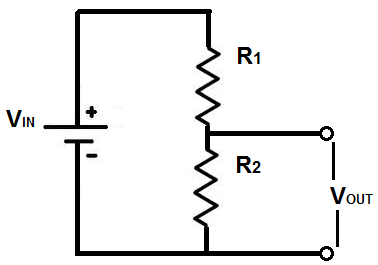If you are using the voltage sensor module that I have used you will notice it has three terminals on the output side, the one marked with a + symbol is not used, I am not sure why it is even there.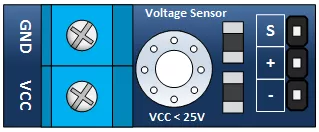DS18b20 Temperature Sensors:
These devices can be used with just two wires but I have used three as it seems this works best in noisy environments. The big advantage of these “one wire” devices is you can have more than one connected to the same input pin on the Arduino. The only requirement is one 4.7k pull-up resistor connected between VDD and DQ. The code I have used is based on the MySensors Dallas Temperature example.

ACS712 Current Sensing Module:

First be aware that the pin out can vary on these modules so check before you connect up. You can see in the picture below that the VCC and GND pins are reversed on these two.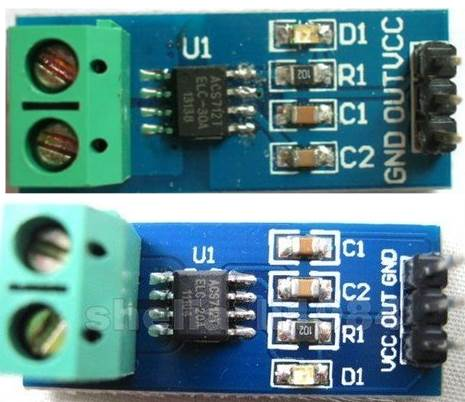The ACS712 current sensing module is a 5v device that measures current flow and returns a voltage that can be used to determine the amount of current flowing. It is available in three versions 5A, 20A and 30A .
Importantly for this application they read current flow in both directions. To do this they output 2.5v when no current is flowing and increase or decrease the voltage depending on which direction the current is flowing.
None of them use the full 0 to 5v range for their output, the 20A version I have used has a sensitivity value of 100mv per 1A of current flow. As this is a 20A device we can multiply the 100mv by 20 and we get 2000mv or a 2v change for 20amps. We know the ACS712 shows an output of 2.5v when no current is flowing so using the 2v we now have the output range from .5v to 4.5v.
To use this device then we need a way to convert its voltage output to a measurement in amps.

Here is the basic equation needed

amps = ((voltsPerCount * count) – offsetVolts) / sensitivityVolts

Where

VoltsPerCount is the volt value per count of the Arduino ADC. The ADC measures the voltage present at the analog pin (0 to 5 volt) and then assigns the result as a count from 0 to 1023, that's 1024 counts including zero. If we divide 5v by 1024 we get .00488 volts for each count. So VoltsPerCount = .00488 Volts

count is the value our Arduino ADC returns after processing the output from the ACS712.

So after the first part of the equation (.00488 * (count) is complete we have converted our count reading from the arduino into volts.

The ACS712 can measure current flow in both directions so it outputs a voltage of 2.5v as it's center point (when no current is flowing). To allow for this offset we must subtract the 2.5v to center a 2.5 voltage reading at zero. So offsetVolts = 2.5 Volts

That's what the next part does ((.00488 * (count)) - 2.5) After this is complete we are left with a negative or positive voltage for current flowing in either direction or a value of zero for no current flow.

The sensitivity values for the ACS712 are shown on the datasheet as

185mv per Amp for the 5A
100mv per Amp for the 20A
66mv per Amp for the 30A

So to convert our value in volts to a value in amps we need to add the last part
((.00488 * (count)) – 2.5)/sensitivityVolts

Where sensitivityVolts equals the sensitivity value in volts for the device you are using. In my case the 20A version 100mv = .1 volts

``````  amps = ((.00488 * (count)) – 2.5) / .1
``````

Another way to do this would be to use the Arduino map function
we worked out earlier that the 20A version would output in the range from .5 to 4.5 volts, to convert that to count values we need to divide by .00488 .
Which gives us .5 / .00488 = 102 and 4.5 / .00488 = 922. map can only work with integers so it is best to use milliamps instead of amps for the output result.

The Arduino code for the 20A ACS712 would be:

``````     milliamps =  map(count, 102, 922, -20000, 20000);
``````

Both of the above methods give very close results so you could use either. I have used the first method as it is easier to select the version of ACS712 you are using by changing the sensitivity.

The ACS712 is a hall effect device so it can be affected by stray magnetic fields that are nearby. It is best to locate it as far away as possible from any magnetic source to ensure you are getting a true reading. In use I found this was sometimes hard to achieve so I have added code to zero the reading if required. To do this I have used a push button and LED. If you are getting a constant current reading when no current is flowing simply push and hold the button until the led flashes. The sketch will then work out the offset required and apply that to zero the reading. This offset amount will then be saved to the Arduino EEPROM and will be reloaded and used even if the arduino is reset.

I am using Domoticz as my controller, here is what the end result looks like.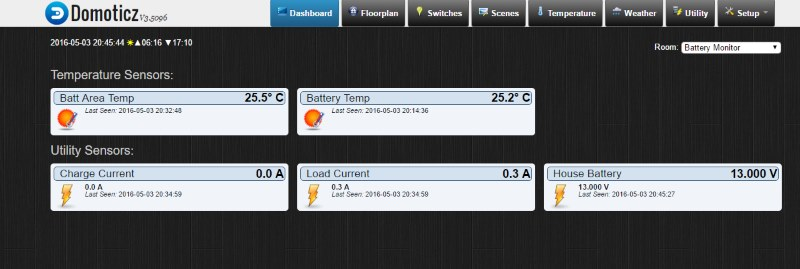• Looking over the schematic you have connected the 5V from the LM2596 directly to the 5V PIN over the arduino.
Is this correct ?
Don't we have to connect it to the VIN PIN??

• @mrc-core from what I understand from https://www.arduino.cc/en/Main/ArduinoBoardNano, VIN wants 6-20V, so 5V should be connected to 5V

• Yes @mrc-core as far as I am aware it is fine to supply 5v directly to this pin. If you have a look at the Nano spec page @mfalkvidd has listed above you will see that under the power heading it says

"The Arduino Nano can be powered via the Mini-B USB connection, 6-20V unregulated external power supply (pin 30), or 5V regulated external power supply (pin 27)."

Pin 27 is the 5v pin so all should be ok. Of course you must take care that you only supply 5v or you may damage the arduino.

While the input voltage for the nano VIN is shown as from 6-20V they recommend keeping within 7-12V , when the solar panel is charging the voltage could at times exceed 14V and from what I have read elsewhere I would expect the nano regulator could get quite warm.

The Lm2596 module has an input voltage of up to 40V and can deliver 2A so will work very well for this application. They are also very cheap so well worth using i think.

• I have made a change to the Sketch. I was saving my data to the same EEPROM positions that MySensors use for their data. So i have now moved well away from that area.

Edit: No I wasn't so no changes were needed

• Here is a picture of the finished board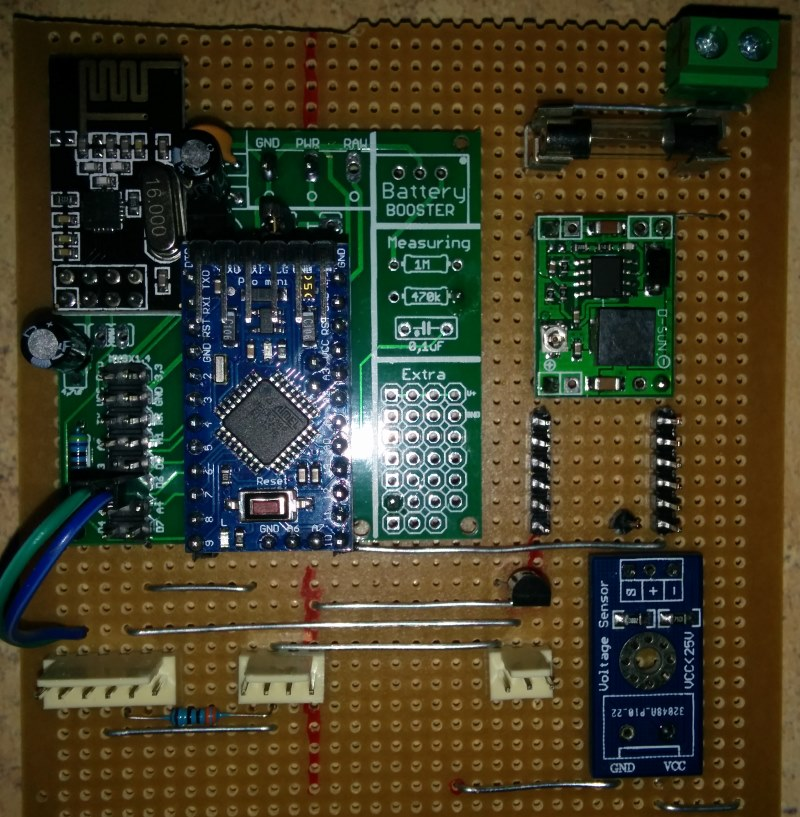I have used the PCB designed by @sundberg84

• @Boots33 said:

I have made a change to the Sketch. I was saving my data to the same EEPROM positions that MySensors use for their data. So i have now moved well away from that area.

Are you're sure, that 514 and 515 are vaild values? API documentation states, that pos parameter in saveState/loadState must be between 0 and 255. AFAIK data stored by saveState are written into a separate section of EEPROM, which doesn't interfere with MySensors own configuration data.

• @googlyeyz thanks for that info. I should have looked closer at the API. I originally used positions. 0 and 1 and thought that would conflict but I will now change back

• Hi.
I'm having some troubles trying to use the project.
I have the solar pannel that goes directly to the solar regulator. In the solar regulator i have the battery connection and the load connection.
In the battery connection i have my 12V battery connected and i have connected the arduinos over the load connection.

My problem is that i dont see were to connect the ACS712 module on the solar regulator.
At this moment i had connect the ACS712 over the load connection in the solar regulator but the values i'm getting are not correct...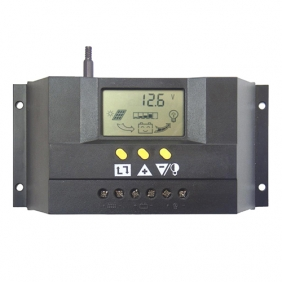Can anyone give me a help to fiz this problem.
Thanks.

• The correct hookup for that type of regulator should look something like the picture below.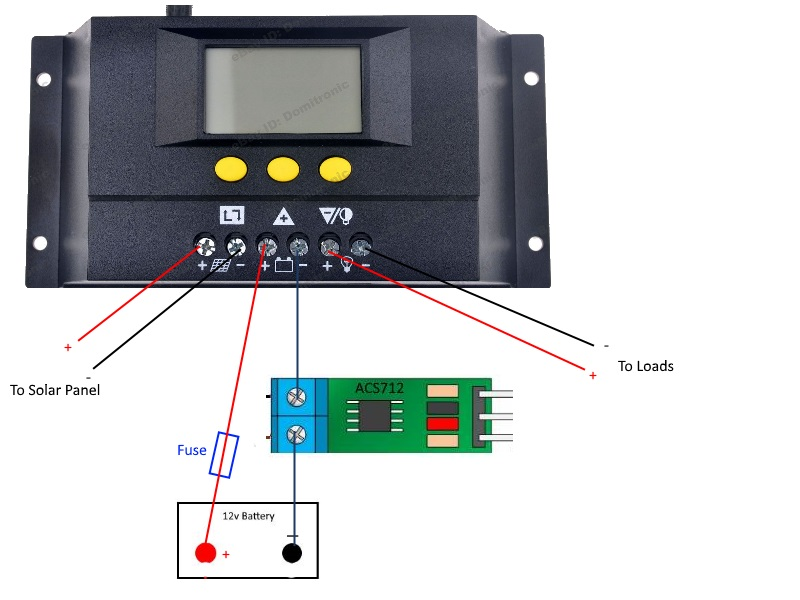Remember in this position the acs712 is reading current to and from the battery only. So if you had a load of 5A and your panel was supplying 3A then your readout would show a discharge of 2A

If your reading is showing a discharge when it should be showing a charge then just reverse the connections at the acs712.

• Thanks for the reply, i'm going to make the changes tonight.
I'm getting readings from 8A to 18A this is not correct.

• How much current were you expecting to see

• @Boots33 To be honest i just thought the values i was getting were too hight since the battery is 12V 20AH and i only have 4 arduino nano with some sensors using the power from the battery.

Today with one day having the changes made like you have said to do, i have value from the battery between hight 8.2A and 6.6A low, but the media value is almost always on 7.4A.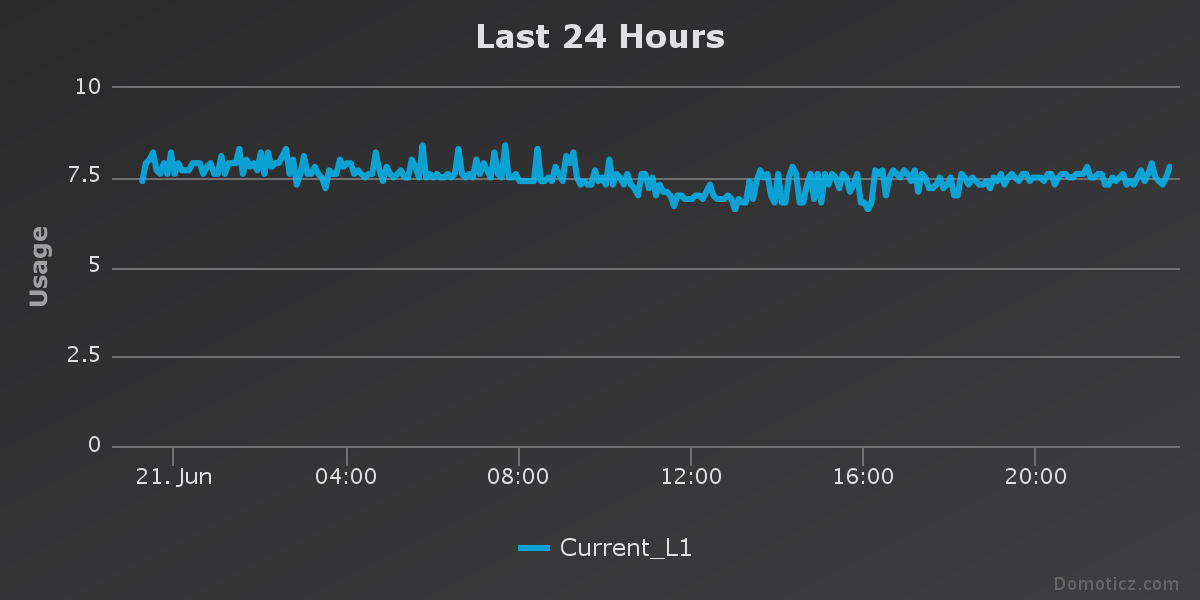On the voltage side i'm getting high value 8.8V and low value 7.8V.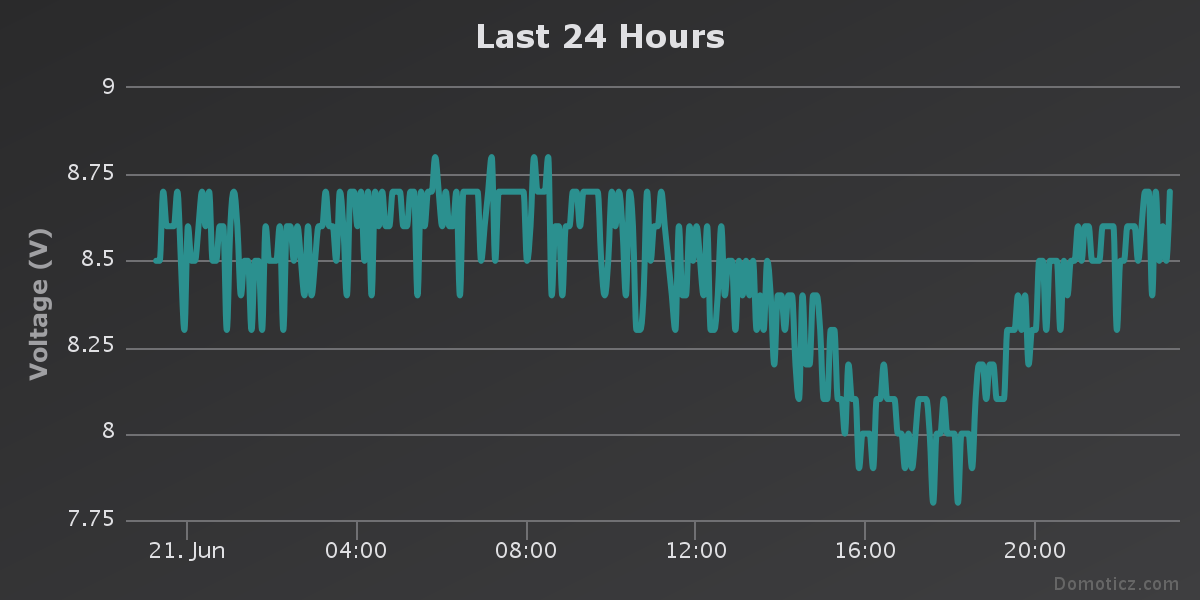Is this good values, correct values for a 12V battery ??

• I don't think those readings are correct. For a 12v battery it should be around 12.7v when full and not being charged. When it is being charged it should be up near 14v. Around 10.9v is considered fully discharged so your readings are way too low for a 12v battery. Your current readings seem too high as well for just a few arduino boards. Can you take some readings with a multimeter to cross check the data. Also check your 5v supply voltage, it is very important for this to be spot on 5v when taking analogue readings.

• @Boots33 Have check the voltage from the battery and is on 12V at this time is discharging the current i couldn't get but tomorrow i'll make new readings and see the values.
The 5V supply voltage is set to 5.02V will have to reduce the 0.02V to get just 5V.

• I should think 5.02v will be close enough. What value resistors are you using for your voltage divider.

• We appear to be operating in different time zones so have posted a couple of things to try.

The first thing to do is re-check all your wiring, I know you probably have done that already but best to make sure anyway.

Then you should try and isolate the cause of your problems.

This line of code in the sketch

``````int voltSenseMax = 25000;
``````

is where you set the maximum input voltage of your voltage divider. If you are not using the 25v voltage sensor module you will need to change the 25000 to suit your voltage divider, by changing that value up or down you can also make adjustments in the voltage reading to fine tune the output. Your reading seems to be out by a few volts so i think you should take a look at the voltage divider first and see if you can locate the reason.

I will show the way to check the 25v module and you can use the same method to work out the values if you have used a different divider.

Because we are using a voltage divider to alter the 12v battery voltage into a 5 volt representation that the Arduino can use we will need to work out what voltage we are expecting to see at pin A0
the formula we need is

``````ArduinoIn = (BatVolts/voltsPerCount25)*voltsPerCount5
``````

first we need the voltsPerCount25 for the 25v divider, so that will be 25/1024 which = .02441

then we need the voltsPerCount5 for the Arduino 5v input 5/1024 which = .00488

BatVolts is the actual battery voltage, you will need to measure this with your multimeter.

So as an example

If you measured your battery and it has 11.3v

(11.3/.02441)*.00488 = 2.26v

So if the voltage divider is working ok we would expect to see a voltage of around 2.3v at the arduino input. Which would = a count of (2.3/.00488) around 471

You can also remove the comments from the two Serial.print lines in this part of the sketch to see what the Arduino is producing

``````// ------------------ Start voltage readings --------------------

sample_count = 0;
sum = 0;
while (sample_count < NUM_SAMPLES) {                                   // take a number of voltage samples
sample_count++;
delay(10);
}
//Serial.print("sum count..."); Serial.println((sum / NUM_SAMPLES));      // print the count result. will be between 0 and 1023
int voltageI = map(sum/NUM_SAMPLES,0,1023,0,voltSenseMax);              // map the reading and get our result in millivolts
//Serial.print("mapped volts..."); Serial.println(voltageI / 1000.0, 1);  // convert millivolts back to volts and print. the 1 at the end determines how many decimal places to show

``````

• Here are what my graphs look like. You can see your voltage should be a bit higher if all is well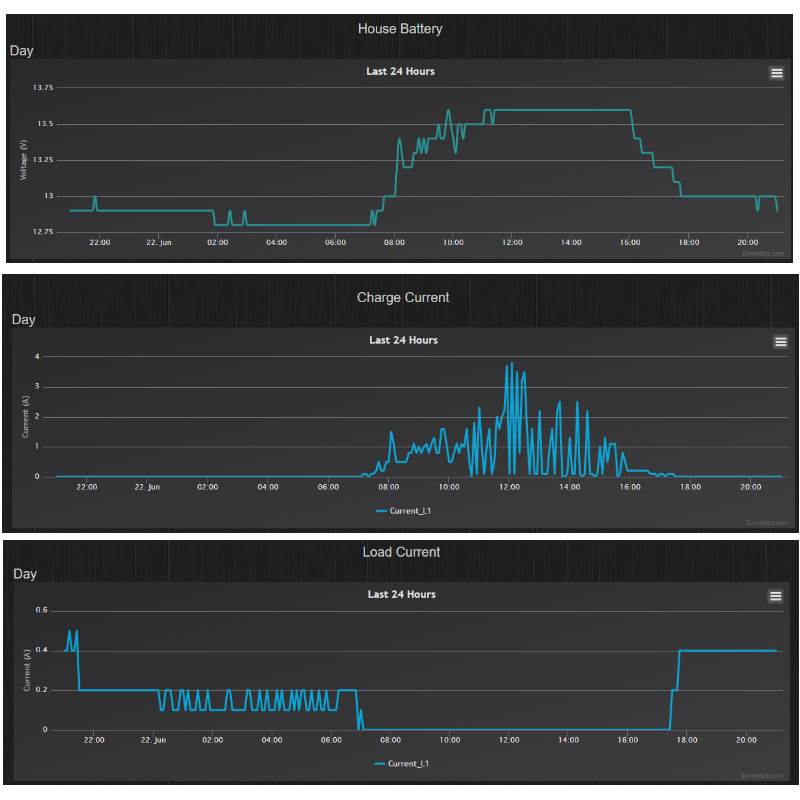• Hi. i have read the post's you have made, one problem i have found its on the voltage sensing module don't now why it's not workin, simply dead, i have already bought 3 other modules and now i'm waiting for them to arrieved hope to get them until friday.

Have seen your graphs and they are really differents from mine.

Once again today with the multimeter i've made some readings from the battery and i got values in 13,2V - 12,9V and this values were measured at 19:00

Once again Thanks for helping me

• @Boots33 said:

30k for R1 and 7.5k for R2

I was going to try to build the voltage sensing but i don't have any 7.5K resistor.... i can put 3 10k Resistors in serie to get the 30k Resistor but i cant make the 7.5K.

Can i use one 10K resistor in the place of the 7.5K, will this work ?? or will the values i'll get be rong??

• Yes that would work. That would give you a maximum input of 20v so you will need to change relevant line in the sketch from 25000 to 20000

You can try different values on this web site. http://www.raltron.com/cust/tools/voltage_divider.asp

• I'm going to try this way and see what values i'll get.
Once again thanks.

• I really don't get this.
Has i can see i did everything correct but i'm still getting weard values.... In the charge regulator that i have the solar30 i'm connecting the ACS712 like you told.
The voltage dividir i had it connected also on the battery vcc for the 30K resistor and ground on the 10K resistor, over the code i ahd change the "voltSenseMax" to 20000.

With this changes the House battery went from 8.4V to 16.2V but when i make the reading off the battery with a multimeter i get 13V and also the charge regulator screen shows 13V...
The charge current is over 0A now but is night at my place ill wait until tomorrow to see if this values change over the morning and day.
The load current is 9.1A this one i dont get it...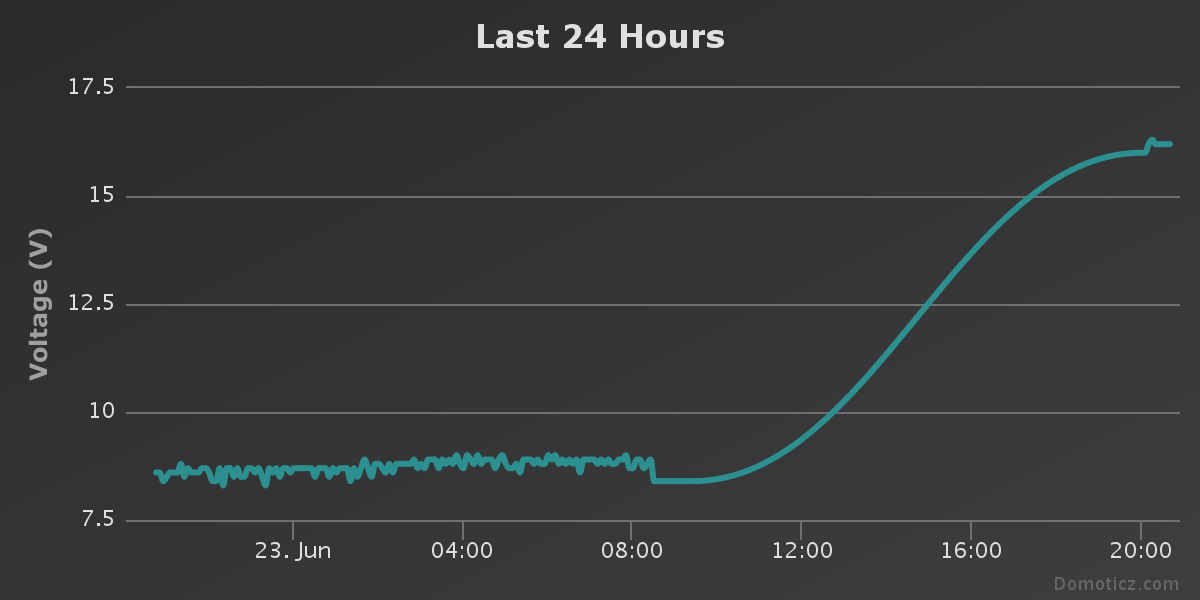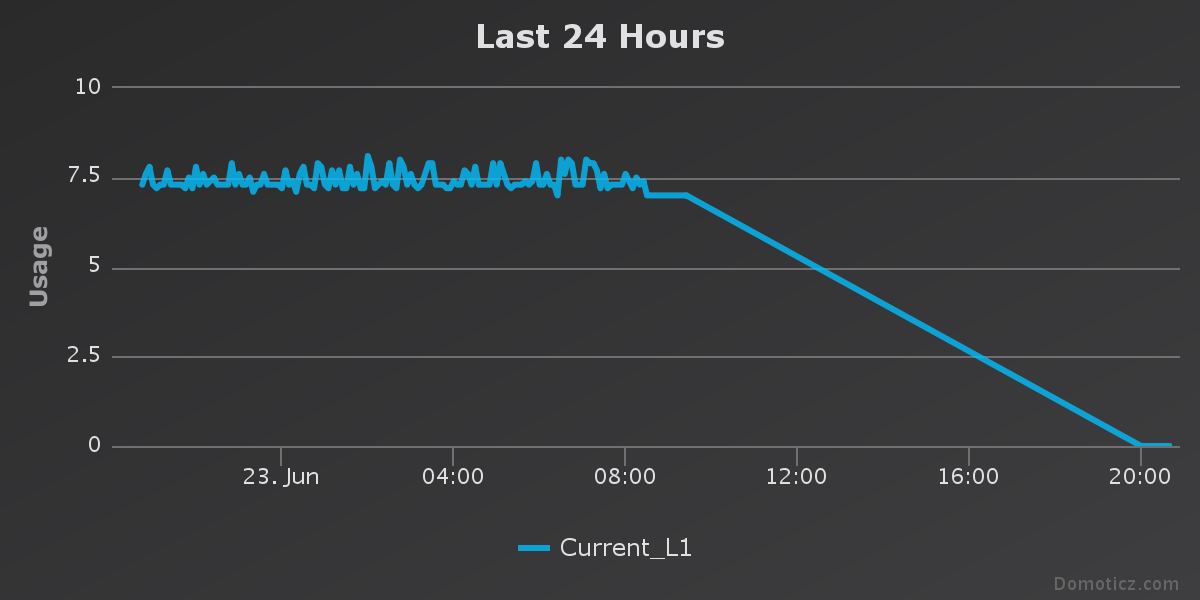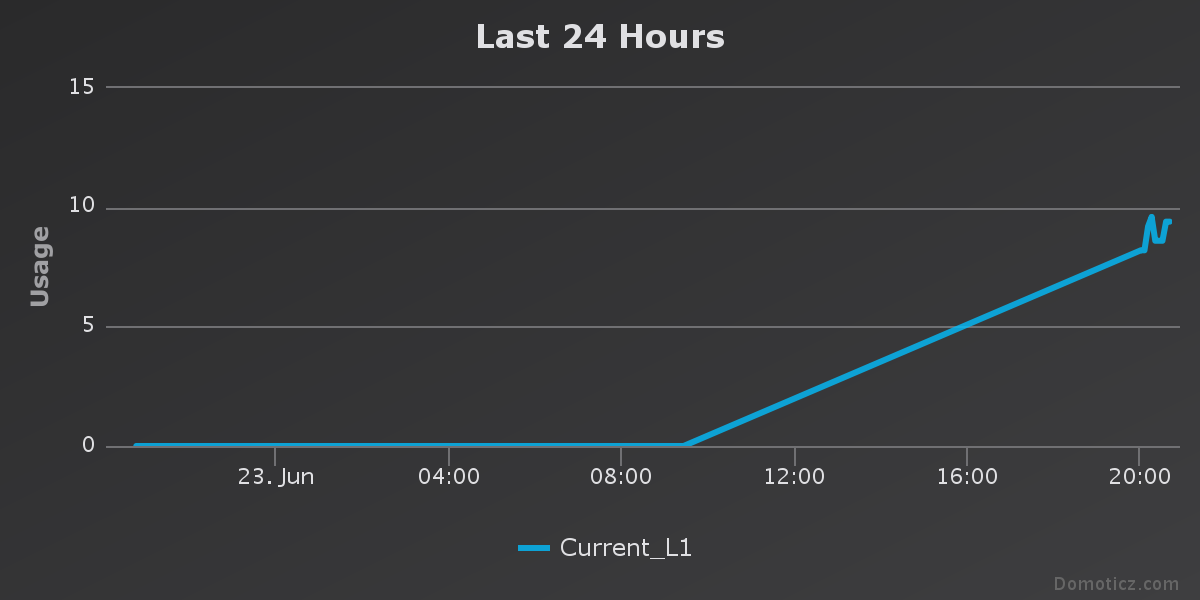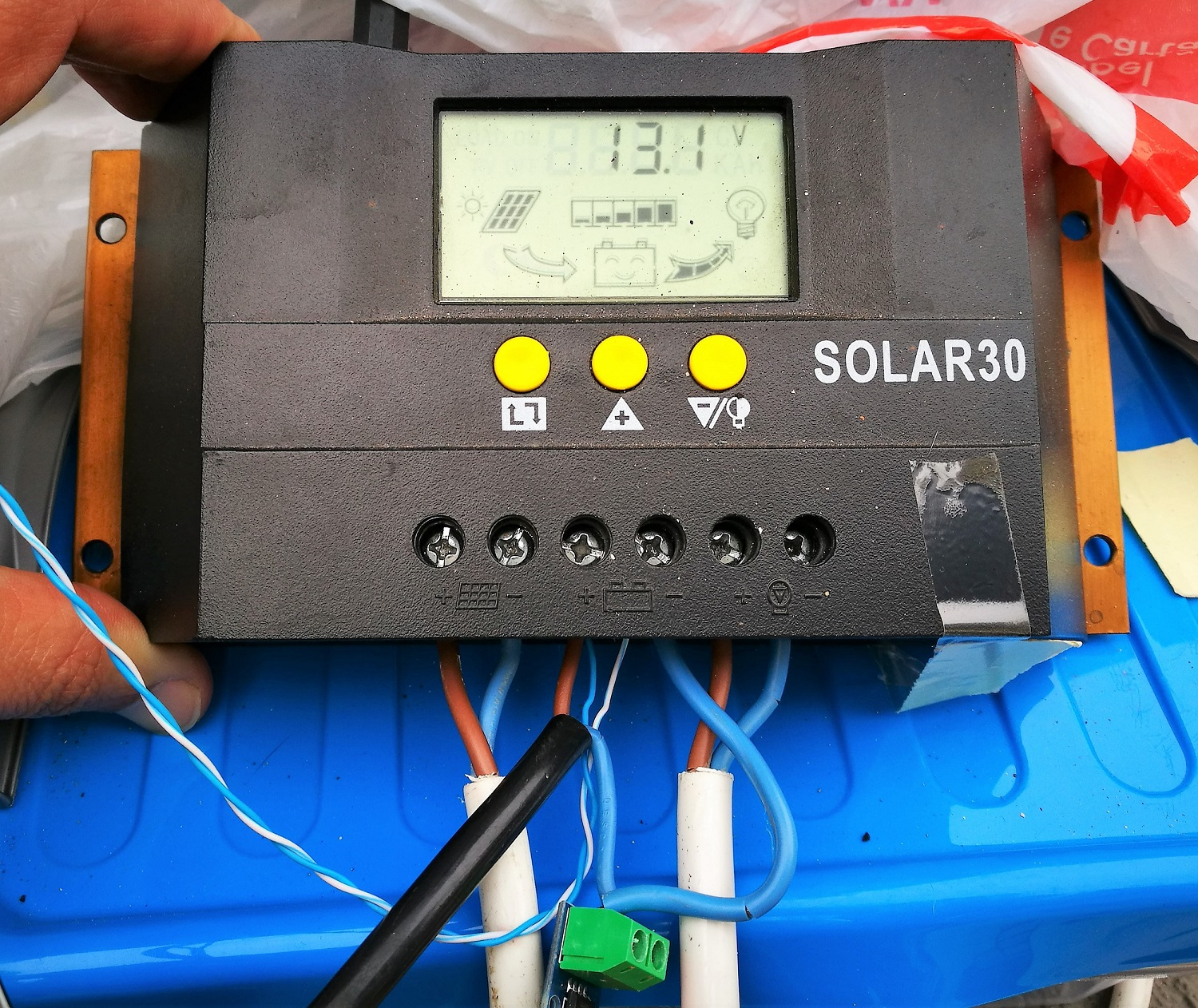• When you were measuring 13v at the battery what was the voltage at pin A0 on the arduino. You will need to compare the readings to see if the divider is working correctly. Have you removed the comments on the serial print lines , you can then check those results with your other measurements. Also check the resistance of the resistors in your voltage divider with your multimeter to make sure they are close to the values you expect.

From what I can see in the picture the asc712 looks to be hooked up OK. They are very sensitive to electromagnetic interference so you may want to try it further away from your regulator.

• Hi.
Ok i did not measure the pin A0 when i was measuring de 13V over the battery but i can do that.I have removed the comments but i hook up the arduino in the battery box and did not look over the serie monitor on PC, once again i'll do that today.
I think the voltage divider i ok, i did a check using a 3,7V battery and the values ia had get with the voltage divider were 0,20% differente from the ones i was getting over the multimeter, 3.2V to 3.4V

I'm going to mak some changes over the place were the asc712 is, i have a box on the wall 40cm to 80cm distance off the batteryes. That's were the ACS712 is going to be.

Thanks.

• Just took the readings from pin A0 and the values are 3.2V when the battery readins says 13V.
The house battery is fix at 16V or 16.2V but i could not move the acs712 to the box i wanted it's night now and i can't see well, sow this change will be done tomorrow. While i'm wainting let's see if this 16V changes during the day off tomorrow.

Well it's being dificult to get this project work like a charm but it's staring to get tune up thank's to your help Boots33.

• I've a mistake when said 4 arduino nano with some sensors, correcting this mistake:
1º arduino nano with UV sensor;
1º arduino nano with lux sensor, pressure sensor, temp/hum sensor and one anemometer; "this is my meteo box sensor"
1º arduino nano with the acs712, voltage sensor and the dallas temperature sensor
1º arduino nano controling a rain gauge.

It's 4 arduino nano with 7 sensors, i don't believe that all this put together are draining 8.6A from the battery. Isn't this a too high value ?

• 3.2v at A0 sounds about right for a 20v divider reading 13v at the battery. So your divider seems to be OK. you now need to check the results from those serial print statements and see how they compare.

The code for the voltage measurement is pretty simple with the map function doing most of the work. Not a lot to go wrong there I think. But we will know more when you get the above results.

8.6A is too high for the current. if you were drawing that much your 20ah battery would be flat in a couple of hours, so the reading is wrong. Can you check with your multimeter and see how much is being drawn. I would not think it would even be 1 amp with just 4 arduino's connected

• With a 3.2v at A0 you should have a count of around 656 from the serial print

• Hi once again.
Today i had to dismount all the solar system my arduino nano just died... don't now why and also the repeater node also dead...

I had replaced the arduino for the solar system i had also put he battery inside my house to make all the tests.
here is the serial monitor log:

Requesting temperatures...
sum count...511
mapped volts...10.0
Milliamps...-95
Requesting temperatures...
sum count...522
mapped volts...10.2
send: 6-6-3-0 s=3,c=1,t=38,pt=7,l=5,sg=0,st=ok:10.2
Milliamps...-95
Requesting temperatures...
sum count...506
mapped volts...9.9
send: 6-6-3-0 s=3,c=1,t=38,pt=7,l=5,sg=0,st=ok:9.9
Milliamps...-95
Requesting temperatures...
sum count...511
mapped volts...10.0
send: 6-6-3-0 s=3,c=1,t=38,pt=7,l=5,sg=0,st=ok:10.0
Milliamps...-95
Requesting temperatures...
sum count...511
mapped volts...10.0
Milliamps...-95
Requesting temperatures...
sum count...511
mapped volts...10.0
Milliamps...-95
Requesting temperatures...
sum count...511
mapped volts...10.0
Milliamps...-95
Requesting temperatures...

One thing i had notice now is that house voltage is 10V but solar regulator and multimeter says 13V, and i had moved the acs712 away from the battery.
The test i made was one CPU fan connect to the solar regulator load, this fan is 12A - 0.25A.
Running the fan the solar regulator shows a Load off 0.1A and the arduino shows values between 0A and 0.3A

send: 6-6-3-0 s=255,c=0,t=17,pt=0,l=5,sg=0,st=ok:1.5.1
send: 6-6-3-0 s=255,c=3,t=6,pt=1,l=1,sg=0,st=ok:3
sensor started, id=6, parent=3, distance=2
send: 6-6-3-0 s=255,c=3,t=11,pt=0,l=21,sg=0,st=ok:Battery Status Sensor
send: 6-6-3-0 s=255,c=3,t=12,pt=0,l=1,sg=0,st=ok:1
send: 6-6-3-0 s=0,c=0,t=6,pt=0,l=0,sg=0,st=fail:
send: 6-6-3-0 s=1,c=0,t=6,pt=0,l=0,sg=0,st=fail:
send: 6-6-3-0 s=3,c=0,t=38,pt=0,l=0,sg=0,st=fail:
send: 6-6-3-0 s=4,c=0,t=39,pt=0,l=0,sg=0,st=fail:
send: 6-6-3-0 s=5,c=0,t=39,pt=0,l=0,sg=0,st=fail:
sum count...511
mapped volts...10.0
send: 6-6-3-0 s=3,c=1,t=38,pt=7,l=5,sg=0,st=fail:10.0
find parent
send: 6-6-255-255 s=255,c=3,t=7,pt=0,l=0,sg=0,st=bc:
Milliamps...52
send: 6-6-3-0 s=4,c=1,t=39,pt=7,l=5,sg=0,st=ok:0.1
send: 6-6-3-0 s=5,c=1,t=39,pt=2,l=2,sg=0,st=fail:0
Requesting temperatures...
send: 6-6-3-0 s=0,c=1,t=0,pt=7,l=5,sg=0,st=fail:30.3
send: 6-6-3-0 s=1,c=1,t=0,pt=7,l=5,sg=0,st=fail:-127.0
sum count...517
mapped volts...10.1
send: 6-6-3-0 s=3,c=1,t=38,pt=7,l=5,sg=0,st=ok:10.1
Milliamps...200
send: 6-6-3-0 s=4,c=1,t=39,pt=7,l=5,sg=0,st=ok:0.2
send: 6-6-3-0 s=5,c=1,t=39,pt=2,l=2,sg=0,st=ok:0
Requesting temperatures...
send: 6-6-3-0 s=0,c=1,t=0,pt=7,l=5,sg=0,st=fail:29.9
sum count...511
mapped volts...10.0
send: 6-6-3-0 s=3,c=1,t=38,pt=7,l=5,sg=0,st=ok:10.0
Milliamps...126
send: 6-6-3-0 s=4,c=1,t=39,pt=7,l=5,sg=0,st=ok:0.1
send: 6-6-3-0 s=5,c=1,t=39,pt=2,l=2,sg=0,st=ok:0
Requesting temperatures...

• OK that is good you can now see the serial print lines. The voltage conversion seems to look ok .... a count of 511 shows a voltage of 10. So now I need you to get all the readings at once

measure battery voltage
measure pin A0 voltage
and show the serial data as you have above

then we can see where the trouble lies

• Battery Voltage - 13.09
pin A0 - 1.055

send: 6-6-3-0 s=255,c=0,t=17,pt=0,l=5,sg=0,st=ok:1.5.1
send: 6-6-3-0 s=255,c=3,t=6,pt=1,l=1,sg=0,st=fail:3
sensor started, id=6, parent=3, distance=2
send: 6-6-3-0 s=255,c=3,t=11,pt=0,l=21,sg=0,st=ok:Battery Status Sensor
send: 6-6-3-0 s=255,c=3,t=12,pt=0,l=1,sg=0,st=fail:1
send: 6-6-3-0 s=0,c=0,t=6,pt=0,l=0,sg=0,st=fail:
send: 6-6-3-0 s=1,c=0,t=6,pt=0,l=0,sg=0,st=ok:
send: 6-6-3-0 s=3,c=0,t=38,pt=0,l=0,sg=0,st=fail:
send: 6-6-3-0 s=4,c=0,t=39,pt=0,l=0,sg=0,st=ok:
send: 6-6-3-0 s=5,c=0,t=39,pt=0,l=0,sg=0,st=fail:
sum count...532
mapped volts...10.4
send: 6-6-3-0 s=3,c=1,t=38,pt=7,l=5,sg=0,st=fail:10.4
Milliamps...347
send: 6-6-3-0 s=4,c=1,t=39,pt=7,l=5,sg=0,st=fail:0.3
send: 6-6-3-0 s=5,c=1,t=39,pt=2,l=2,sg=0,st=fail:0
Requesting temperatures...
send: 6-6-3-0 s=0,c=1,t=0,pt=7,l=5,sg=0,st=fail:29.5
send: 6-6-3-0 s=1,c=1,t=0,pt=7,l=5,sg=0,st=ok:-127.0
sum count...513
mapped volts...10.0
send: 6-6-3-0 s=3,c=1,t=38,pt=7,l=5,sg=0,st=ok:10.0
Milliamps...347
Requesting temperatures...
send: 6-6-3-0 s=0,c=1,t=0,pt=7,l=5,sg=0,st=ok:29.4
sum count...519
mapped volts...10.1
send: 6-6-3-0 s=3,c=1,t=38,pt=7,l=5,sg=0,st=ok:10.1
Milliamps...421
send: 6-6-3-0 s=4,c=1,t=39,pt=7,l=5,sg=0,st=ok:0.4
send: 6-6-3-0 s=5,c=1,t=39,pt=2,l=2,sg=0,st=ok:0
Requesting temperatures...
send: 6-6-3-0 s=0,c=1,t=0,pt=7,l=5,sg=0,st=fail:29.5
sum count...511
mapped volts...10.0
send: 6-6-3-0 s=3,c=1,t=38,pt=7,l=5,sg=0,st=ok:10.0
Milliamps...347
send: 6-6-3-0 s=4,c=1,t=39,pt=7,l=5,sg=0,st=ok:0.3
send: 6-6-3-0 s=5,c=1,t=39,pt=2,l=2,sg=0,st=ok:0
Requesting temperatures...
send: 6-6-3-0 s=0,c=1,t=0,pt=7,l=5,sg=0,st=fail:29.4
sum count...511
mapped volts...10.0
Milliamps...347
Requesting temperatures...
send: 6-6-3-0 s=0,c=1,t=0,pt=7,l=5,sg=0,st=ok:29.5
sum count...511
mapped volts...10.0
Milliamps...200
send: 6-6-3-0 s=4,c=1,t=39,pt=7,l=5,sg=0,st=ok:0.2
send: 6-6-3-0 s=5,c=1,t=39,pt=2,l=2,sg=0,st=ok:0
Requesting temperatures...
sum count...485
mapped volts...9.5
send: 6-6-3-0 s=3,c=1,t=38,pt=7,l=5,sg=0,st=ok:9.5
Milliamps...273
send: 6-6-3-0 s=4,c=1,t=39,pt=7,l=5,sg=0,st=ok:0.3
send: 6-6-3-0 s=5,c=1,t=39,pt=2,l=2,sg=0,st=ok:0
Requesting temperatures...
sum count...510
mapped volts...10.0
send: 6-6-3-0 s=3,c=1,t=38,pt=7,l=5,sg=0,st=ok:10.0
Milliamps...347
send: 6-6-3-0 s=4,c=1,t=39,pt=7,l=5,sg=0,st=ok:0.3
send: 6-6-3-0 s=5,c=1,t=39,pt=2,l=2,sg=0,st=fail:0
Requesting temperatures...
sum count...501
mapped volts...9.8
send: 6-6-3-0 s=3,c=1,t=38,pt=7,l=5,sg=0,st=ok:9.8
Milliamps...347
Requesting temperatures...
sum count...511
mapped volts...10.0
send: 6-6-3-0 s=3,c=1,t=38,pt=7,l=5,sg=0,st=ok:10.0
Milliamps...347
Requesting temperatures...

Once again i had connect the 12v fan to the load of the charge regulator

• If you are still using the 20v divider, with a battery voltage of 13.09 you should have a voltage of 3.27 at pin A0

1.055v is way too low, check you connections to make sure they are tight. measure the voltage at the divider input to make sure the 13v is there as well.

post results again

• 3.29v It's the value i'm getting at the end off the 20v divider but at pin A0 i have 1.23V

Requesting temperatures...
sum count...198
mapped volts...3.9
send: 6-6-3-0 s=3,c=1,t=38,pt=7,l=5,sg=0,st=ok:3.9
Milliamps...200
send: 6-6-3-0 s=4,c=1,t=39,pt=7,l=5,sg=0,st=ok:0.2
send: 6-6-3-0 s=5,c=1,t=39,pt=2,l=2,sg=0,st=ok:0
Requesting temperatures...
sum count...511
mapped volts...10.0
send: 6-6-3-0 s=3,c=1,t=38,pt=7,l=5,sg=0,st=ok:10.0
Milliamps...126
send: 6-6-3-0 s=4,c=1,t=39,pt=7,l=5,sg=0,st=ok:0.1
send: 6-6-3-0 s=5,c=1,t=39,pt=2,l=2,sg=0,st=ok:0
Requesting temperatures...
sum count...511
mapped volts...10.0
Milliamps...-21
send: 6-6-3-0 s=4,c=1,t=39,pt=2,l=2,sg=0,st=ok:0
send: 6-6-3-0 s=5,c=1,t=39,pt=7,l=5,sg=0,st=fail:0.0
Requesting temperatures...
sum count...520
mapped volts...10.2
send: 6-6-3-0 s=3,c=1,t=38,pt=7,l=5,sg=0,st=ok:10.2
Milliamps...126
send: 6-6-3-0 s=4,c=1,t=39,pt=7,l=5,sg=0,st=ok:0.1
send: 6-6-3-0 s=5,c=1,t=39,pt=2,l=2,sg=0,st=fail:0
Requesting temperatures...
sum count...533
mapped volts...10.4
send: 6-6-3-0 s=3,c=1,t=38,pt=7,l=5,sg=0,st=ok:10.4
Milliamps...52
send: 6-6-3-0 s=4,c=1,t=39,pt=7,l=5,sg=0,st=ok:0.1
send: 6-6-3-0 s=5,c=1,t=39,pt=2,l=2,sg=0,st=ok:0
Requesting temperatures...
sum count...511
mapped volts...10.0
send: 6-6-3-0 s=3,c=1,t=38,pt=7,l=5,sg=0,st=ok:10.0
Milliamps...-21
send: 6-6-3-0 s=4,c=1,t=39,pt=2,l=2,sg=0,st=ok:0
send: 6-6-3-0 s=5,c=1,t=39,pt=7,l=5,sg=0,st=ok:0.0
Requesting temperatures...
sum count...511
mapped volts...10.0
Milliamps...200
send: 6-6-3-0 s=4,c=1,t=39,pt=7,l=5,sg=0,st=ok:0.2
send: 6-6-3-0 s=5,c=1,t=39,pt=2,l=2,sg=0,st=ok:0
Requesting temperatures...

• then that is where the problem may be. the output of the divider should be connected to A0 so they should be the same. look at the diagram below• I think the problem is fix now.

bad cable between ground from 20v divider and arduino ground.
now i'm getting the same value at pin a0

here are the new logs

send: 6-6-3-0 s=255,c=3,t=11,pt=0,l=21,sg=0,st=ok:Battery Status Sensor
send: 6-6-3-0 s=255,c=3,t=12,pt=0,l=1,sg=0,st=ok:1
send: 6-6-3-0 s=0,c=0,t=6,pt=0,l=0,sg=0,st=ok:
send: 6-6-3-0 s=1,c=0,t=6,pt=0,l=0,sg=0,st=fail:
send: 6-6-3-0 s=3,c=0,t=38,pt=0,l=0,sg=0,st=fail:
send: 6-6-3-0 s=4,c=0,t=39,pt=0,l=0,sg=0,st=fail:
send: 6-6-3-0 s=5,c=0,t=39,pt=0,l=0,sg=0,st=ok:
sum count...715
mapped volts...14.0
send: 6-6-3-0 s=3,c=1,t=38,pt=7,l=5,sg=0,st=ok:14.0
Milliamps...-21
send: 6-6-3-0 s=4,c=1,t=39,pt=2,l=2,sg=0,st=ok:0
send: 6-6-3-0 s=5,c=1,t=39,pt=7,l=5,sg=0,st=ok:0.0
Requesting temperatures...
send: 6-6-3-0 s=0,c=1,t=0,pt=7,l=5,sg=0,st=ok:29.3
send: 6-6-3-0 s=1,c=1,t=0,pt=7,l=5,sg=0,st=ok:-127.0
sum count...710
mapped volts...13.9
send: 6-6-3-0 s=3,c=1,t=38,pt=7,l=5,sg=0,st=ok:13.9
Milliamps...52
send: 6-6-3-0 s=4,c=1,t=39,pt=7,l=5,sg=0,st=ok:0.1
send: 6-6-3-0 s=5,c=1,t=39,pt=2,l=2,sg=0,st=ok:0
Requesting temperatures...
sum count...713
mapped volts...13.9
send: 6-6-3-0 s=3,c=1,t=38,pt=7,l=5,sg=0,st=ok:13.9
Milliamps...-21
send: 6-6-3-0 s=4,c=1,t=39,pt=2,l=2,sg=0,st=ok:0
send: 6-6-3-0 s=5,c=1,t=39,pt=7,l=5,sg=0,st=ok:0.0
Requesting temperatures...
sum count...711
mapped volts...13.9
send: 6-6-3-0 s=3,c=1,t=38,pt=7,l=5,sg=0,st=ok:13.9
Milliamps...200
send: 6-6-3-0 s=4,c=1,t=39,pt=7,l=5,sg=0,st=ok:0.2
send: 6-6-3-0 s=5,c=1,t=39,pt=2,l=2,sg=0,st=ok:0
Requesting temperatures...
sum count...712
mapped volts...13.9
send: 6-6-3-0 s=3,c=1,t=38,pt=7,l=5,sg=0,st=ok:13.9
Milliamps...200
Requesting temperatures...
sum count...709
mapped volts...13.9
send: 6-6-3-0 s=3,c=1,t=38,pt=7,l=5,sg=0,st=ok:13.9
Milliamps...126
send: 6-6-3-0 s=4,c=1,t=39,pt=7,l=5,sg=0,st=ok:0.1
send: 6-6-3-0 s=5,c=1,t=39,pt=2,l=2,sg=0,st=ok:0
Requesting temperatures...
sum count...713
mapped volts...13.9
send: 6-6-3-0 s=3,c=1,t=38,pt=7,l=5,sg=0,st=ok:13.9
Milliamps...126
Requesting temperatures...

still using the cpu fan 12v 0.25A pin A0 3.28V
Mapped volts 13.9 it's very near the 13.2V the multimeter gives at the battery.
This is the best results i had until now.

Thanks boots33 for all your help.

• Great to hear you have found the problem. If the voltages are not quite correct you can adjust the 20000 value up or down a bit as needed to fine tune the output result.

You can use these to check for the problem

for a 20v divider

20/1024 = .019531

to get the count that should be shown by serial print...: batteryvoltage / .019531

That means you should see a count of 670 from the serial print

to get the voltage that should be at pin A0...: count * .00488

670 * .00488 = 3.27v

to get the map result

20000/1024 = 19.531mv or .01953v

so 670 * .01953 = 13.1v

• The best way to setup the acs712 is to put it where it is going to be mounted but have no current going through it. (you can just disconnect one power lead) it should show a reading close to zero.
If not push the button and hold till the led flashes (may take a while depending on how much delay you are using) once the led flashes you can let go of the button and the offset to zero the reading will be applied. This offset will also be saved in the arduino eeprom so it will be reloaded if the arduino is rebooted etc.
then the readings should be very close to zero.
you can now reconnect and it should give you the correct reading.

• Hallo

Mein Englisch ist nicht so gut.
Ich habe versucht das Projekt nach zu bauen. Den Button brauche ich (denke ich) nicht, dafür habe ich das script jedoch um 2 weitere current und voltage Sensoren erweitert. Das Ziel ist 3 mal Current und 3 mal Volt als Ergebnis zu bekommen. Ich habe 30A Sensoren und einen 30A Laderegler. Dazwischen sind jeweils 30A Sicherungen.
Leider kommt in Domoticz aber nur ein mal Volt und 2 mal Current an. Leider habe ich auch nicht wirklich viel Ahnung vom Programmieren. Könnte hier mal jemand über den Sketch gucken um zu sehen ob ich einen Fehler darin habe?

``````/*Sketch for a MySensor node to monitor a 12v battery with a solar panel for charging
* The node monitors battery voltage,current into and out of the battery, ambient temperature and battery temperature.
* 2 x DS18b20 dallas temperature ic's their data pins connected to arduino digital pin 3
* 1 x ACS712 current sensor module connected to  arduino analog pin A4
* 1 x 25v voltage sensor module connected to arduino analog pin A0
* 1 x nRF24L01+  2.4ghz tranceiver connected as per the MySensors web site.
* 1 x LED connected via a 330 ohm resistor to pin 6
* 1 x push button connected to pin 5
*/

#include <MySensor.h>
#include <SPI.h>
#include <OneWire.h>
#include <DallasTemperature.h>

#define ONE_WIRE_BUS 3                       // Ds18b20 data wire is connected to digital pin 3 on the Arduino
#define ID_S_TEMPA 0                         // First temp device
#define ID_S_TEMPB 1                         // second temp device
#define ID_S_MULTIMETERV1 3                   // Multimeter device for voltage measurement
#define ID_S_MULTIMETERC1 4                   // Multimeter device for positive current measurement
#define ID_S_MULTIMETERC11 5                  // Multimeter device for negative current measurement
#define ID_S_MULTIMETERV2 3                   // Multimeter device for voltage measurement
#define ID_S_MULTIMETERC2 4                   // Multimeter device for positive current measurement
#define ID_S_MULTIMETERC12 5                  // Multimeter device for negative current measurement
#define ID_S_MULTIMETERV3 6                   // Multimeter device for voltage measurement
#define ID_S_MULTIMETERC3 7                   // Multimeter device for positive current measurement
#define ID_S_MULTIMETERC13 8
#define NUM_SAMPLES 10                       // number of analog voltage samples to take per reading

int ledPin = 6;                               // the pin for the LED
int buttonPin = 5;                            // the input pin for offset pushbutton
int buttonState = 0;                          // variable for reading the pin status
unsigned long SLEEP_TIME = 30000;            // Sleep time between reads (in milliseconds)
int lastmilli1 = 25000;                       // set to an arbitary number outside of expected current sensor range to ensure a change when first run
int lastmilli2 = 25000;                       // set to an arbitary number outside of expected current sensor range to ensure a change when first run
int lastmilli3 = 25000;                       // set to an arbitary number outside of expected current sensor range to ensure a change when first run
float sensitivity = 66 ;                    //change this to 185 for ACS712-5 or to 100 for ACS712-20A or to 66 for ACS712-30A
int VQ = 0;                                  //Placeholder for quiescent voltage calculations
int ACSPin1 = A4;                             // Analog pin number the ACS712 data pin connects to
int ACSPin2 = A5;                             // Analog pin number the ACS712 data pin connects to
int ACSPin3 = A3;                             // Analog pin number the ACS712 data pin connects to
float lastTemperature;                    //Array to hold the last temp readings sent to gateway, only send new data if different
int sum = 0;                                 // sum of voltage samples taken
unsigned char sample_count = 0;              // current sample number
int lastVoltage1 = 30000;                     // set to an arbitary number outside of expected voltage sensor range to ensure a change when first run
int lastVoltage2 = 30000;                     // set to an arbitary number outside of expected voltage sensor range to ensure a change when first run
int lastVoltage3 = 30000;                     // set to an arbitary number outside of expected voltage sensor range to ensure a change when first run
int voltagePin1 = A0;                         // analog pin voltage sensor or voltage divider is connected to
int voltagePin2 = A1;                         // analog pin voltage sensor or voltage divider is connected to
int voltagePin3 = A2;                         // analog pin voltage sensor or voltage divider is connected to
int voltSense1Max = 25000;                    // set to the maximum input voltage in millivolts of your voltage divider input
int voltSense2Max = 25000;                    // set to the maximum input voltage in millivolts of your voltage divider input
int voltSense3Max = 25000;                    // set to the maximum input voltage in millivolts of your voltage divider input
OneWire oneWire(ONE_WIRE_BUS);               // Setup a oneWire instance to communicate with any OneWire devices (not just Maxim/Dallas temperature ICs)
DallasTemperature sensors(&oneWire);         // Pass our oneWire reference to Dallas Temperature.

MySensor gw;

// ------ Initialize  messages -------
MyMessage msg(0,V_TEMP);
MyMessage msg_S_MULTIMETERv1(ID_S_MULTIMETERV1,V_VOLTAGE);
MyMessage msg_S_MULTIMETERc1(ID_S_MULTIMETERC1,V_CURRENT);
MyMessage msg_S_MULTIMETERc11(ID_S_MULTIMETERC11,V_CURRENT);
MyMessage msg_S_MULTIMETERv2(ID_S_MULTIMETERV2,V_VOLTAGE);
MyMessage msg_S_MULTIMETERc2(ID_S_MULTIMETERC2,V_CURRENT);
MyMessage msg_S_MULTIMETERc12(ID_S_MULTIMETERC12,V_CURRENT);
MyMessage msg_S_MULTIMETERv3(ID_S_MULTIMETERV3,V_VOLTAGE);
MyMessage msg_S_MULTIMETERc3(ID_S_MULTIMETERC3,V_CURRENT);
MyMessage msg_S_MULTIMETERc13(ID_S_MULTIMETERC13,V_CURRENT);

void setup()
{

sensors.begin();                                    // Start up the onewire library
gw.begin();                                         // Startup and initialize MySensors library. Set callback for incoming messages.
gw.sendSketchInfo("Battery Status Sensor", "1");    // Send the sketch version information to the gateway and Controller

// ------ Present all sensors to controller ------
gw.present(ID_S_TEMPA, S_TEMP);
gw.present(ID_S_TEMPB, S_TEMP);
gw.present(ID_S_MULTIMETERV1,V_VOLTAGE);
gw.present(ID_S_MULTIMETERC1,V_CURRENT);
gw.present(ID_S_MULTIMETERC11,V_CURRENT);
gw.present(ID_S_MULTIMETERV2,V_VOLTAGE);
gw.present(ID_S_MULTIMETERC2,V_CURRENT);
gw.present(ID_S_MULTIMETERC12,V_CURRENT);
gw.present(ID_S_MULTIMETERV3,V_VOLTAGE);
gw.present(ID_S_MULTIMETERC3,V_CURRENT);
gw.present(ID_S_MULTIMETERC13,V_CURRENT);

pinMode(buttonPin, INPUT_PULLUP);                     // Set buttonPin as input and turn on internal pull up resistor
pinMode(ledPin, OUTPUT);                              // Set ledPin as output
digitalWrite(ledPin, LOW);                            // Make sure ledPin is off

// ------ load offset for current sensor
if (validCheck == 120){                          // check to see if valid data exists
//  Serial.print(" positive VQ offset loaded..."); Serial.println(VQ);
}
else if (validCheck == 125) {
//  Serial.print(" negative VQ offset loaded..."); Serial.println(VQ);
}
else {
// Serial.println("VQ offset not set");
}

delay(500);
}

void loop()
{

//Serial.print("buttonstate..."); Serial.println(buttonState);
if (buttonState == LOW) {
VQ = determineVQ(ACSPin1);                           //Returns the offset count needed to show zero with no load

if (VQ >= 0 && VQ < 255) {                              //check for valid data. VQ is positive number
gw.saveState(0, 120);                               // Store 120 value  in eeprom position 0. use this to check for valid data at boot
gw.saveState(1, VQ);                                // Store offset count in eeprom. in case of re-boot
}
else if (VQ < 0 && VQ > -255) {                              // VQ is a negative number. negatives cannot be stored in eeprom
gw.saveState(0, 125);                               // Store 125 value  in eeprom position 0. use this to check for valid data at boot
gw.saveState(1, abs(VQ));                                // convert VQ to positive and  Store offset count in eeprom. in case of re-boot
}

}

// ------------------ Start voltage 1 readings --------------------

sample_count = 0;
sum = 0;
while (sample_count < NUM_SAMPLES) {                                   // take a number of voltage samples
sample_count++;
delay(10);
}
//Serial.print("sum count..."); Serial.println((sum / NUM_SAMPLES));      // print the count result. will be between 0 and 1023
int voltage1I = map(sum/NUM_SAMPLES,0,1023,0,voltSense1Max);              // map the reading and get our result in millivolts
//Serial.print("mapped volts..."); Serial.println(voltageI / 1000.0, 1);  // convert millivolts back to volts and print. the 1 at the end determines how many decimal places to show

if ( voltage1I != lastVoltage1) {                                         // check if we have a new value. only send data if it is different
gw.send(msg_S_MULTIMETERv1.set(voltage1I / 1000.0, 1));                  // voltagel is in millivolts so we divide by 1000 to convert back to volts and
// send voltage message to gateway with 1 decimal place
lastVoltage1 = voltage1I;                                                // copy the current voltage reading for testing on the next loop
}

int milli1 = readCurrent(ACSPin1);                                       // take a reading from the ACS712 and send to the readcurrent function

//Serial.print("Milliamps..."); Serial.println(milli);                   // print the value (in milliamps) returned

if ( milli1 != lastmilli1)                                               // check if value has changed
{
if ( milli1 > 0)                                                       // Battery is charging
{
gw.send(msg_S_MULTIMETERc1.set(milli1/1000.0, 1));                     // Send new data to charging amp device
gw.send(msg_S_MULTIMETERc11.set(0));                                  // set the dis-charging amp device to zero
lastmilli1 =  milli1;
}
else if (milli1 < 0)                                                  // Battery is discharging
{
gw.send(msg_S_MULTIMETERc1.set(0));                                  // set the charging amp device to zero
gw.send(msg_S_MULTIMETERc11.set(abs(milli1)/1000.0, 1));             //  use abs(milli) to Send a positive number  to dis-charging amp device
lastmilli1 =  milli1;
}
else                                                                // No current flowing, set both to zero
{
gw.send(msg_S_MULTIMETERc1.set(0));
gw.send(msg_S_MULTIMETERc11.set(0));
lastmilli1 =  milli1;
}
}

Serial.println(" Requesting temperatures...");

// Fetch temperatures from Dallas sensors
sensors.requestTemperatures();                  // call sensors.requestTemperatures() to issue a global temperature request to all devices on the bus

// ------- query conversion time and sleep until conversion completed ------
int16_t conversionTime = sensors.millisToWaitForConversion(sensors.getResolution());
gw.sleep(conversionTime);

for (int i=0; i<2; i++) {
//  Serial.print("Temperature for Device: ");Serial.print(i);Serial.print(" is: ");
// Serial.println(sensors.getTempCByIndex(i)); // Why "byIndex"?
// You can have more than one IC on the same bus.
// 0 refers to the first IC on the wire

float temperature = static_cast<float>(static_cast<int>((sensors.getTempCByIndex(i)) * 10.)) / 10.;  // Fetch and round temperature to one decimal in celcius

if (lastTemperature[i] != temperature)               // check for a changed temperature reading
{
gw.send(msg.setSensor(i).set(temperature,1));     // Send in the new temperature
lastTemperature[i]=temperature;                   // Save new temperatures for next compare
}
}

// ------------------ Start 2 voltage readings --------------------

sample_count = 0;
sum = 0;
while (sample_count < NUM_SAMPLES) {                                   // take a number of voltage samples
sample_count++;
delay(10);
}
//Serial.print("sum count..."); Serial.println((sum / NUM_SAMPLES));      // print the count result. will be between 0 and 1023
int voltage2I = map(sum/NUM_SAMPLES,0,1023,0,voltSense2Max);              // map the reading and get our result in millivolts
//Serial.print("mapped volts..."); Serial.println(voltageI / 1000.0, 1);  // convert millivolts back to volts and print. the 1 at the end determines how many decimal places to show

if ( voltage2I != lastVoltage2) {                                         // check if we have a new value. only send data if it is different
gw.send(msg_S_MULTIMETERv2.set(voltage2I / 1000.0, 1));                  // voltagel is in millivolts so we divide by 1000 to convert back to volts and
// send voltage message to gateway with 1 decimal place
lastVoltage2 = voltage2I;                                                // copy the current voltage reading for testing on the next loop
}

int milli2 = readCurrent(ACSPin2);                                       // take a reading from the ACS712 and send to the readcurrent function

//Serial.print("Milliamps..."); Serial.println(milli);                   // print the value (in milliamps) returned

if ( milli2 != lastmilli2)                                               // check if value has changed
{
if ( milli2 > 0)                                                       // Battery is charging
{
gw.send(msg_S_MULTIMETERc2.set(milli2/1000.0, 1));                     // Send new data to charging amp device
gw.send(msg_S_MULTIMETERc12.set(0));                                  // set the dis-charging amp device to zero
lastmilli2 =  milli2;
}
else if (milli1 < 0)                                                  // Battery is discharging
{
gw.send(msg_S_MULTIMETERc2.set(0));                                  // set the charging amp device to zero
gw.send(msg_S_MULTIMETERc12.set(abs(milli2)/1000.0, 1));             //  use abs(milli) to Send a positive number  to dis-charging amp device
lastmilli2 =  milli2;
}
else                                                                // No current flowing, set both to zero
{
gw.send(msg_S_MULTIMETERc2.set(0));
gw.send(msg_S_MULTIMETERc12.set(0));
lastmilli2 =  milli2;
}
}

// ------------------ Start voltage 3 readings --------------------

sample_count = 0;
sum = 0;
while (sample_count < NUM_SAMPLES) {                                   // take a number of voltage samples
sample_count++;
delay(10);
}
//Serial.print("sum count..."); Serial.println((sum / NUM_SAMPLES));      // print the count result. will be between 0 and 1023
int voltage3I = map(sum/NUM_SAMPLES,0,1023,0,voltSense3Max);              // map the reading and get our result in millivolts
//Serial.print("mapped volts..."); Serial.println(voltageI / 1000.0, 1);  // convert millivolts back to volts and print. the 1 at the end determines how many decimal places to show

if ( voltage3I != lastVoltage3) {                                         // check if we have a new value. only send data if it is different
gw.send(msg_S_MULTIMETERv3.set(voltage3I / 1000.0, 1));                  // voltagel is in millivolts so we divide by 1000 to convert back to volts and
// send voltage message to gateway with 1 decimal place
lastVoltage3 = voltage3I;                                                // copy the current voltage reading for testing on the next loop
}

int milli3 = readCurrent(ACSPin3);                                       // take a reading from the ACS712 and send to the readcurrent function

//Serial.print("Milliamps..."); Serial.println(milli);                   // print the value (in milliamps) returned

if ( milli3 != lastmilli3)                                               // check if value has changed
{
if ( milli3 > 0)                                                       // Battery is charging
{
gw.send(msg_S_MULTIMETERc3.set(milli3/1000.0, 1));                     // Send new data to charging amp device
gw.send(msg_S_MULTIMETERc13.set(0));                                  // set the dis-charging amp device to zero
lastmilli3 =  milli3;
}
else if (milli3 < 0)                                                  // Battery is discharging
{
gw.send(msg_S_MULTIMETERc3.set(0));                                  // set the charging amp device to zero
gw.send(msg_S_MULTIMETERc13.set(abs(milli3)/1000.0, 1));             //  use abs(milli) to Send a positive number  to dis-charging amp device
lastmilli3 =  milli3;
}
else                                                                // No current flowing, set both to zero
{
gw.send(msg_S_MULTIMETERc3.set(0));
gw.send(msg_S_MULTIMETERc13.set(0));
lastmilli3 =  milli3;
}
}
gw.sleep(SLEEP_TIME);
}

/*-------------- Function to get the offset required for ACS712 to show zero with no current flowing -----------------*/
int determineVQ(int PIN)
{
digitalWrite(ledPin, HIGH);                                      // Turn on LED to indicate offset being calculated
delay(500);                                                      // Delay to hold LED on
digitalWrite(ledPin, LOW);                                       // Turn off LED
delay(150);                                                      // Delay to let readings stabilise
//  Serial.print("estimating avg. quiscent voltage:");
long acsCount = 0;
for (int i=0; i<5000; i++)                                       //read 5000 samples to stabilise value
{
acsCount += analogRead(PIN);                                   // read the count value between 0 and 1023 and add it to acsCount
delay(1);
}
acsCount /= 5000;                                                      // acsCount now eaquals the average of the 5000 readings taken
//  Serial.print(map(acsCount, 0, 1023, 0, 5000));Serial.println(" mV");   //Print the avg in millivolts
//  Serial.print("acsCount:");Serial.println(acsCount);                               //Print the actual count value

return int(acsCount - 512);                                            // return the count difference. 512 is the count for 2.5v which is what the reading should be with no current flow

}

/*--------------- Function to read current flowing ------------------*/

{
int count = 0;
for (int i=0; i<5; i++)                                        //read 5 analog count samples to stabilise value
{
count += analogRead(PIN) - VQ;                               //subtract the offset count VQ to improve accuracy
delay(1);
//  Serial.print("raw count..."); Serial.println(count);
}
/* Notes on the conversion below
*  .00488 is the volt value per count of the arduino adc. The analog pin measures from 0 to 5 volt and then assigns the result to
*  a count from 0 to 1023, thats 1024 counts including zero. If we devide 5v by 1024 we get .oo488 volts for each count.
*
*  The (count/5) just gets us the average of our 5 count samples.
*
*  So after the first part of the equation  (.00488 * (count/5) is complete we have converted our count reading into volts.
*
*  The ACS712 can measure current flow in both directions so it outputs a voltage of  2.5v as it's center point (when no current is flowing).
*  To allow for this offset we must subtract the 2.5v to center our voltage reading.
*
* Thats what the next part does (.00488 * (count/5)) - 2.5) After this is complete we are left with either a negative or  positive voltage
*
* NOTE: While the ACS712 is a 5v device it does not use the full 0 to 5v for it's output. The datasheet shows the 20A version has a sensitivity of
*  100mv per amp, so if we multiply 100mv by 20 we get 2v.  That means the 20A ACS712 has an output range from .5v to 4.5v.
*
* So to convert our reading in volts to a reading in amps we need to add the last part ((.00488 * (count/5)) - 2.5)/(sensitivity/1000).
* The variable sensitivity is defined at the begining of the sketch and holds the ACS712 sensitvity amount, it is stored in millivolts.
* That is 66mv for the 30amp,  100mv for the 20amp and 185mv for the 5amp. As sensitivity is in millivolts we need to devide it by 1000
* to convert it back to volts so we can use it in the equation.
*
* Now we have our Amps value stored in the float amps. Integers are much easier to work with when checking for zero so we multiply by 1000
* to convert it to milliamps and return it as an integer.
*/

//Serial.print("VQ = ..."); Serial.println(VQ);
//Serial.print("current count..."); Serial.println(count/5);
//Serial.print("map  milliamps..."); Serial.println(map((count/5), 102, 922, -20000, 20000));
float amps = ((.00488 * (count/5)) - 2.5) / (sensitivity/1000);
// Serial.print("float amps..."); Serial.println(amps, 1);
return int (amps * 1000);                                         // convert to milliamps and return as an integer

}
Insert Code Here
``````

English:

Hello

My English is not that good.
I tried to build the project. The button I need (I think) not, but I have extended the script, however, by 2 more current and voltage sensors. The goal is to get 3 times Current and 3 times Volt as a result. I have 30A sensors and a 30A charger. In between are 30A fuses.
Unfortunately in Domoticz but only one time Volt and 2 times Current. Unfortunately I have not really much idea of ​​the programming. Could someone here over the sketch look around to see if I have a mistake in it?do i

Do you have a log of the data being sent from the node? What mysensors library version are you using?

• Hi. i have been working on this solar code and have made the changes to work over mysensors 2.1.1
I'm now triyng to add a line code to monitor the voltage from the solar panels. Can anyone give me a help on this?
I want to now the voltage watts or kws produce over my pannels. The idea is to have domoticz monitoring all data produce over the solar pannel.
Thanks

``````/*Sketch for a MySensor node to monitor a 12v battery with a solar panel for charging
* The node monitors battery voltage,current into and out of the battery, ambient temperature and battery temperature.
* 2 x DS18b20 dallas temperature ic's their data pins connected to arduino digital pin 3
* 1 x ACS712 current sensor module connected to  arduino analog pin A4
* 1 x 25v voltage sensor module connected to arduino analog pin A0
* 1 x nRF24L01+  2.4ghz tranceiver connected as per the MySensors web site.
* 1 x LED connected via a 330 ohm resistor to pin 6
* 1 x push button connected to pin 5
*/

// Enable debug prints to serial monitor
#define MY_DEBUG

// Enable and select radio type attached

// Enable repeater functionality for this node
#define MY_REPEATER_FEATURE

#include <MySensors.h>
#include <SPI.h>
#include <OneWire.h>
#include <DallasTemperature.h>

#define ONE_WIRE_BUS 3                            // Ds18b20 data wire is connected to digital pin 3 on the Arduino
#define ID_S_TEMP_A 0                             // First temp device
#define ID_S_TEMP_B 1                             // Second temp device
#define ID_S_MULTIMETERV 3                        // Multimeter device for voltage measurement
#define ID_S_MULTIMETERC 4                        // Multimeter device for positive current measurement
#define ID_S_MULTIMETERC1 5                       // Multimeter device for negative current measurement
#define NUM_SAMPLES 10                            // number of analog voltage samples to take per reading

int ledPin = 6;                                   // the pin for the LED
int buttonPin = 5;                                // the input pin for offset pushbutton
int buttonState = 0;                              // variable for reading the pin status
unsigned long SLEEP_TIME = 30000;                 // Sleep time between reads (in milliseconds)
int lastmilli = 25000;                            // set to an arbitary number outside of expected current sensor range to ensure a change when first run
float sensitivity = 66 ;                          // change this to 185 for ACS712-5 or to 100 for ACS712-20A or to 66 for ACS712-30A
int VQ = 0;                                       // Placeholder for quiescent voltage calculations
int ACSPin = A4;                                  // Analog pin number the ACS712 data pin connects to
float lastTemperature;                         // Array to hold the last temp readings sent to gateway, only send new data if different
int sum = 0;                                      // sum of voltage samples taken
unsigned char sample_count = 0;                   // current sample number
int lastVoltage = 30000;                          // set to an arbitary number outside of expected voltage sensor range to ensure a change when first run
int voltagePin = A0;                              // analog pin voltage sensor or voltage divider is connected to
int voltSenseMax = 25000;                         // set to the maximum input voltage in millivolts of your voltage divider input
OneWire oneWire(ONE_WIRE_BUS);                    // Setup a oneWire instance to communicate with any OneWire devices (not just Maxim/Dallas temperature ICs)
DallasTemperature sensors(&oneWire);              // Pass our oneWire reference to Dallas Temperature.

// ------ Initialize  messages ------- //
MyMessage msg(0,V_TEMP);
MyMessage msg_S_MULTIMETERv(ID_S_MULTIMETERV,V_VOLTAGE);
MyMessage msg_S_MULTIMETERc(ID_S_MULTIMETERC,V_CURRENT);
MyMessage msg_S_MULTIMETERc1(ID_S_MULTIMETERC1,V_CURRENT);

void presentation()
{
// Send the sketch version information to the gateway
sendSketchInfo("Battery Status Sensor", "2.1.1");

// Register all sensors to gw (they will be created as child devices)
present(ID_S_TEMP_A, S_TEMP);
present(ID_S_TEMP_B, S_TEMP);
present(ID_S_MULTIMETERV,V_VOLTAGE);
present(ID_S_MULTIMETERC,V_CURRENT);
present(ID_S_MULTIMETERC1,V_CURRENT);
}

void setup()
{
sensors.begin();                                      // Start up the onewire library
pinMode(buttonPin, INPUT_PULLUP);                     // Set buttonPin as input and turn on internal pull up resistor
pinMode(ledPin, OUTPUT);                              // Set ledPin as output
digitalWrite(ledPin, LOW);                            // Make sure ledPin is off

// ------ load offset for current sensor
if (validCheck == 120)                                // check to see if valid data exists
{
//Serial.print(" positive VQ offset loaded..."); Serial.println(VQ);
}
else if (validCheck == 125)
{
//Serial.print(" negative VQ offset loaded..."); Serial.println(VQ);
}
else
{
//Serial.println("VQ offset not set");
}
delay(500);
}

void loop()
{
//Serial.print("buttonstate..."); Serial.println(buttonState);
if (buttonState == LOW)
{
VQ = determineVQ(ACSPin);                           // Returns the offset count needed to show zero with no load
if (VQ >= 0 && VQ < 255)
{                                                   // check for valid data. VQ is positive number
saveState(0, 120);                                // Store 120 value  in eeprom position 0. use this to check for valid data at boot
saveState(1, VQ);                                 // Store offset count in eeprom. in case of re-boot
}
else if (VQ < 0 && VQ > -255)                       // VQ is a negative number. negatives cannot be stored in eeprom
{
saveState(0, 125);                                // Store 125 value  in eeprom position 0. use this to check for valid data at boot
saveState(1, abs(VQ));                            // convert VQ to positive and  Store offset count in eeprom. in case of re-boot
}
}

sample_count = 0;
sum = 0;
while (sample_count < NUM_SAMPLES)                     // take a number of voltage samples
{
sample_count++;
delay(10);
}

//Serial.print("sum count..."); Serial.println((sum / NUM_SAMPLES));        // print the count result. will be between 0 and 1023
int voltageI = map(sum/NUM_SAMPLES,0,1023,0,voltSenseMax);                  // map the reading and get our result in millivolts
//Serial.print("mapped volts..."); Serial.println(voltageI / 1000.0, 1);    // convert millivolts back to volts and print. the 1 at the end determines how many decimal places to show

if ( voltageI != lastVoltage)                                               // check if we have a new value. only send data if it is different
{
send(msg_S_MULTIMETERv.set(voltageI / 1000.0, 1));                        // voltagel is in millivolts so we divide by 1000 to convert back to volts and send voltage message to gateway with 1 decimal place
lastVoltage = voltageI;                                                   // copy the current voltage reading for testing on the next loop
}

int milli = readCurrent(ACSPin);                                            // take a reading from the ACS712 and send to the readcurrent function
//Serial.print("Milliamps..."); Serial.println(milli);                      // print the value (in milliamps) returned
if ( milli != lastmilli)                                                    // check if value has changed
{
if ( milli > 0)                                                           // Battery is charging
{
send(msg_S_MULTIMETERc.set(milli/1000.0, 1));                           // Send new data to charging amp device
send(msg_S_MULTIMETERc1.set(0));                                        // set the dis-charging amp device to zero
lastmilli =  milli;
}
else if (milli < 0)                                                       // Battery is discharging
{
send(msg_S_MULTIMETERc.set(0));                                         // set the charging amp device to zero
send(msg_S_MULTIMETERc1.set(abs(milli)/1000.0, 1));                     //  use abs(milli) to Send a positive number  to dis-charging amp device
lastmilli =  milli;
}
else                                                                      // No current flowing, set both to zero
{
send(msg_S_MULTIMETERc.set(0));
send(msg_S_MULTIMETERc1.set(0));
lastmilli =  milli;
}
}

Serial.println(" Requesting temperatures...");
// Fetch temperatures from Dallas sensors
sensors.requestTemperatures();                              // call sensors.requestTemperatures() to issue a global temperature request to all devices on the bus

// ------- query conversion time and sleep until conversion completed ------
int16_t conversionTime = sensors.millisToWaitForConversion(sensors.getResolution());
sleep(conversionTime);

for (int i=0; i<2; i++)
{
//Serial.print("Temperature for Device: ");Serial.print(i);Serial.print(" is: ");
//Serial.println(sensors.getTempCByIndex(i)); // Why "byIndex"?
// You can have more than one IC on the same bus.
// 0 refers to the first IC on the wire

float temperature = static_cast<float>(static_cast<int>((sensors.getTempCByIndex(i)) * 10.)) / 10.;    // Fetch and round temperature to one decimal in celcius
if (lastTemperature[i] != temperature)                                                                 // check for a changed temperature reading
{
send(msg.setSensor(i).set(temperature,1));                                                           // Send in the new temperature
lastTemperature[i]=temperature;                                                                      // Save new temperatures for next compare
}
}
sleep(SLEEP_TIME);
}

/*-------------- Function to get the offset required for ACS712 to show zero with no current flowing -----------------*/
int determineVQ(int PIN)
{
digitalWrite(ledPin, HIGH);                                             // Turn on LED to indicate offset being calculated
delay(500);                                                             // Delay to hold LED on
digitalWrite(ledPin, LOW);                                              // Turn off LED
delay(150);                                                             // Delay to let readings stabilise
//Serial.print("estimating avg. quiscent voltage:");
long acsCount = 0;
for (int i=0; i<5000; i++)                                              // read 5000 samples to stabilise value
{
acsCount += analogRead(PIN);                                          // read the count value between 0 and 1023 and add it to acsCount
delay(1);
}
acsCount /= 5000;                                                       // acsCount now eaquals the average of the 5000 readings taken
//Serial.print(map(acsCount, 0, 1023, 0, 5000));Serial.println(" mV");  // Print the avg in millivolts
//Serial.print("acsCount:");Serial.println(acsCount);                   // Print the actual count value
return int(acsCount - 512);                                             // return the count difference. 512 is the count for 2.5v which is what the reading should be with no current flow

}

/*--------------- Function to read current flowing ------------------*/
{
int count = 0;
for (int i=0; i<5; i++)                                                  // read 5 analog count samples to stabilise value
{
count += analogRead(PIN) - VQ;                                         // subtract the offset count VQ to improve accuracy
delay(1);
//Serial.print("raw count..."); Serial.println(count);
}

/* Notes on the conversion below
*  .00488 is the volt value per count of the arduino adc. The analog pin measures from 0 to 5 volt and then assigns the result to
*  a count from 0 to 1023, thats 1024 counts including zero. If we devide 5v by 1024 we get .oo488 volts for each count.
*
*  The (count/5) just gets us the average of our 5 count samples.
*
*  So after the first part of the equation  (.00488 * (count/5) is complete we have converted our count reading into volts.
*
*  The ACS712 can measure current flow in both directions so it outputs a voltage of  2.5v as it's center point (when no current is flowing).
*  To allow for this offset we must subtract the 2.5v to center our voltage reading.
*
* Thats what the next part does (.00488 * (count/5)) - 2.5) After this is complete we are left with either a negative or  positive voltage
*
* NOTE: While the ACS712 is a 5v device it does not use the full 0 to 5v for it's output. The datasheet shows the 20A version has a sensitivity of
*  100mv per amp, so if we multiply 100mv by 20 we get 2v.  That means the 20A ACS712 has an output range from .5v to 4.5v.
*
* So to convert our reading in volts to a reading in amps we need to add the last part ((.00488 * (count/5)) - 2.5)/(sensitivity/1000).
* The variable sensitivity is defined at the begining of the sketch and holds the ACS712 sensitvity amount, it is stored in millivolts.
* That is 66mv for the 30amp,  100mv for the 20amp and 185mv for the 5amp. As sensitivity is in millivolts we need to devide it by 1000
* to convert it back to volts so we can use it in the equation.
*
* Now we have our Amps value stored in the float amps. Integers are much easier to work with when checking for zero so we multiply by 1000
* to convert it to milliamps and return it as an integer.
*/

//Serial.print("VQ = ..."); Serial.println(VQ);
//Serial.print("current count..."); Serial.println(count/5);
//Serial.print("map  milliamps..."); Serial.println(map((count/5), 102, 922, -20000, 20000));
float amps = ((.00488 * (count/5)) - 2.5) / (sensitivity/1000);
//Serial.print("float amps..."); Serial.println(amps, 1);
return int (amps * 1000);                                         // convert to milliamps and return as an integer
}`````````

• I'm working again on this system but i'm having some troubles... The code has you caan see i have already adapted it to version 2.1.1 Now on the hardware i'm building i have this:

• 1 solar panel 10w connected to the LM2596 that converts the 12v to 5.5v that goes to the LiPo Enhanced Charger Module, here i have the 3,7v bat connected and the arduinos connected to the other power out.

My problem is that when i have the system online the values i'm getting are not right. For the battery voltage i get 5,5v when i believe i should be getting 3,7v or 4,1v maximum.
The charge and load are always on 0,2A or 0,0A

I have the acs712 connected to the negative side off the battery and the Voltage sensor connected to the negative and positive side off the battery.

What i'm i doing rong here ????

• @mrc-core From your code it looks like you are using a 30A acs712 and you are still using the 25v sensor to measure the expected 4v. With only a 10w panel you might be better off with a 5A acs712 and also a voltage divider that maxes out at around 10v. This will give you a better resolution to work with right from the start.

How are you powering the arduino? Perhaps you can do a drawing of how your project is wired together. Remember the arduino needs to have a stable 5v supply to have any chance of giving a good result.

• Hi. sorry for my late replay....
Here's the way i'm powering things up.
I don't have a solar charge since last summer we had a big fire on my island and all my arduino and sensors that were outside were all damage from the fire and hot temperatures. So to fiz this problem i'm using the LM2596 to reduce the 12V from the solar panels to 5.5V so that the lipo charger can work. Above the 5v this charger will get damage.

Some data from this charger:
LED: Red = Charging, Green = Complete
Input Voltage: 4.5 to 5.5V
Battery Max Charge Voltage: 4.2V - charges a 1S Lithium Ion Polymer Battery

This outputs a 4.2V on the load and batt connections.

My arduino and sensors are connected to the load pins from the lipo charger as you can see on the picture.
Thanks for your replay and help.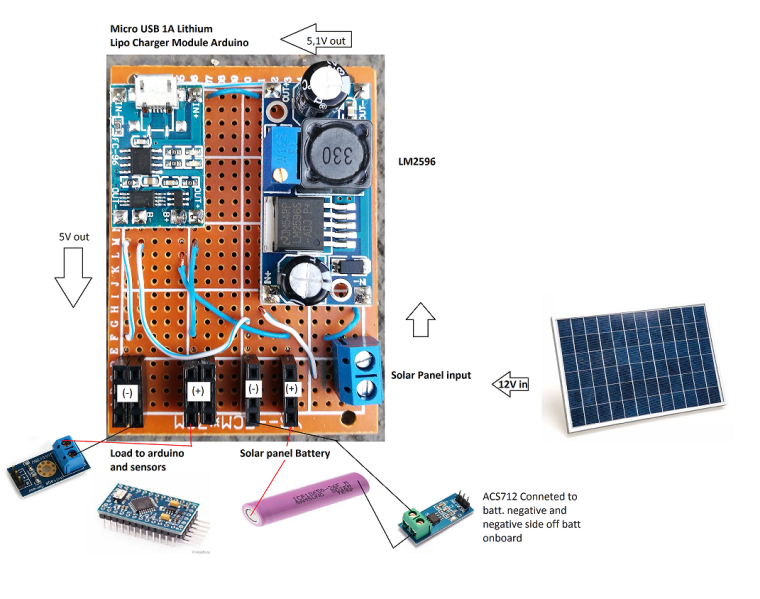• @mrc-core The original circuit/sketch was designed for a 5v arduino and was measuring a 12v system . From your drawing it looks like you will be using a 3v arduino so at the very least you will need to change the voltage divider to give you a better resolution. As to the acs712 it is a 5v device so most likely will not even work on 3v so you may need to source some other device for current measurement. Again you would want a low current device if you are to have any chance of measuring the few milliamps you would be seeing. perhaps a google search for acs712 on 3v will produce a result.

• @Boots33 I'm going to change the arduino mini for an arduino nano to have the 5V still have one in the house.
Acs712 have already buy 2 off them just in case if i have to go back to arduino mini.

I was thinking on booting all my outside arduinos from this solar panel i have 2 more off them that i can connect.

• @Boots33 I have change to an arduino nano but still get some strange values. I'm going to make some changes over this project and see again what ill get.

Thanks for all the help.

• @mrc-core Ok, let me know how you get on.
I think solar powering the nodes is a great idea, I have several nodes and three raspberry pi's running on my house solar system at the moment.

• I just finished setting up this sensor to monitor my solar panels. The voltage sensors is reporting correct, but i'm not sure if the current sensor is right or wired correctly.

This is how it is currently wired.Is this going to give me both charge and discharge?

Thanks!

• Me neither. This is what it looks like to me: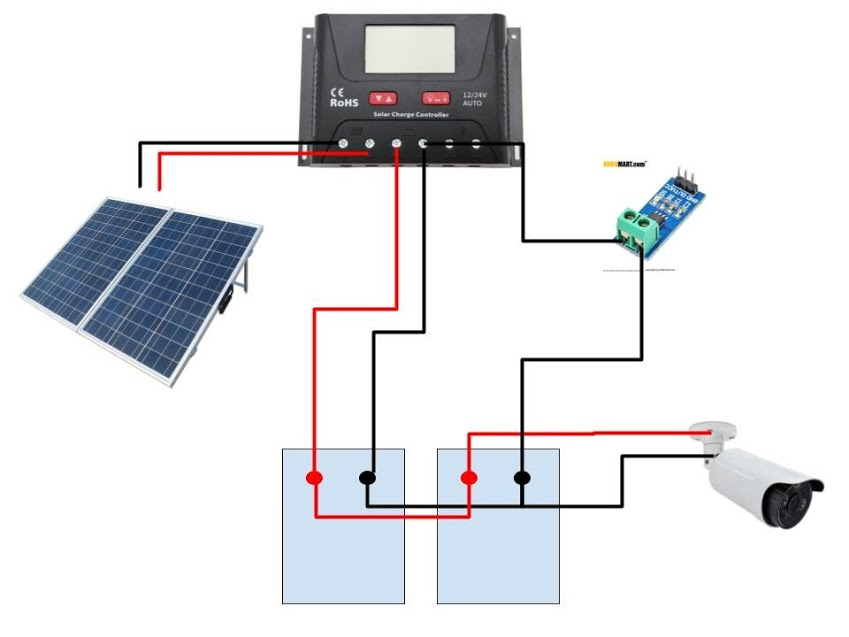• Yes i can see your wiring now and no you are not likely to get any sort of reading with that circuit. You have the 712 wired in parallel with the charge wire, it needs to be in series instead. The load needs to be connected to the same side that the charge wire is on. So a quick re-draw of your circuit would look like this.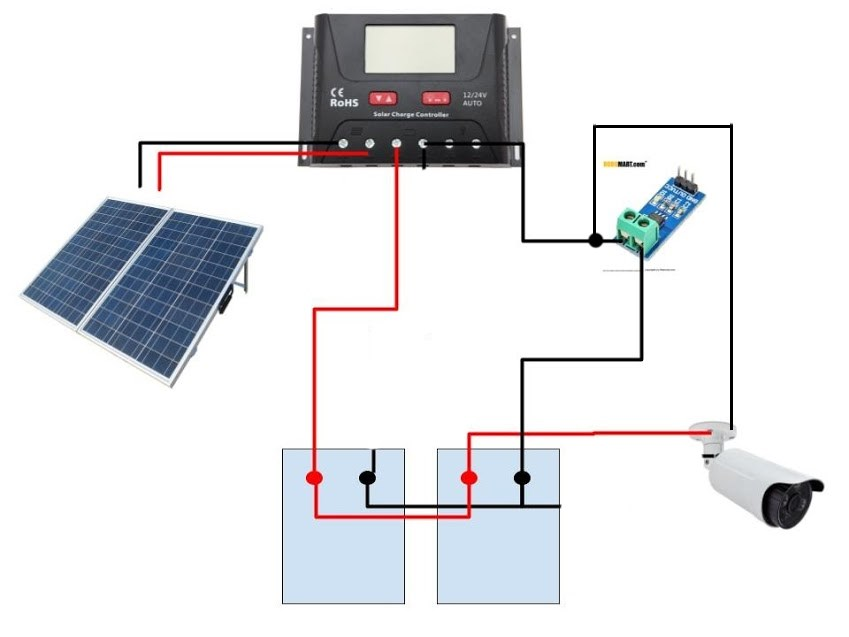Don't forget to add a fuse at the battery positive.

The original drawing from earlier in this thread would also be ok• Okay. I will rewire it this weekend. Thanks!

• Get the sensor rewired yesterday and i am now getting the correct readings! Thanks again for the help.

• @unfadingpyro Great to hear you got it all working. Always a good feeling when a project comes together